# A statistical perspective on year-to-date metrics

Jerry Ratcliffe, and now more recently Jeff Asher, have written about how volatile early year projection of year-to-date (YTD) percent changes. I am going to write about this is not the right way to frame the problem in my opinion – I will present a better behaved estimate that is less volatile, but clearly doesn’t give police departments what they want.

Going to the end advice first – people find me irksome for the suggestion, but you shouldn’t be using percent changes at all. A simple alternative I have stated for low count crime data is a Poisson Z-score, which is simply `2*(sqrt(Current) - sqrt(Past))` – a value of greater than 3 or 4 is a signal the two processes are significantly different (under the null hypothesis that the counts have a Poisson distribution).

## A Better YTD estimate

So here I am going to present a more accurate YTD percent change metric – but don’t take that as advice you should be using YTD percent change. It is more of an exercise to say why you shouldn’t be using this metric to begin with. Year end percent change is defined as:

``(Current - Past)/Past = % Change``

Note that you can rewrite this as:

``````Current/Past - Past/Past  = % Change
Current/Past - 1          = % Change``````

So really it is only the ratio of `Current/Past` that we care about estimating, the translating to a percent doesn’t matter. In the above equations, I am writing these as cumulative totals for the whole year. So lets do breakdowns via subscripts, and shorten Current and Past to C and P respectively. So say we have data through January, people typically estimate the YTD percent change then as:

``(C_January - P_January)/P_January = % Change January``

To make it easier, I am going to write `e` subscript for early, and `l` subscript for later. So if we then estimate YTD for February, we then have `C_January + C_February = C_e`. Also note that `C_e + C_l = Current`, the early observed values plus the later unobserved values equals the year totals. This identifies a clear error when people use only subsets of the data to do YTD year end projections (what both Jerry and Jeff did in their posts to argue against early YTD estimates). You should not just use `P_e` in your estimate, you should use the full prior year counts.

Lets go back to our year end estimate, writing in early/later form:

``[C_e + C_l - (P_e + P_l)]/(P_e + P_l) = % Change``

This only has one unknown in the equation – `C_l`, the unknown rest of year projection. You should not use `(C_e - P_e)/P_e`, as this introduces several stochastic elements where none are needed. `P_e` is not necessarily a good estimate of `P_e + P_l`. So lets do a simple example, imagine we had homicide totals:

``````     Past Current
Jan    2     1
Feb    0
Mar    1
Apr    1
May    1
Jun    1
Jul    1
Aug    1
Sep    1
Oct    1
Nov    1
Dec    1
---
Tot   12``````

The naive way of doing YTD estimates, we would say our January YTD estimates are `(1 - 2)/2 = -50%`. Whereas I am saying, you should use `(1 + C_l)/12` – filling in whatever value you project to the rest of the year totals `C_l`. Simple ones you can do in a spreadsheet are ‘no change’, just fill in the prior year which here would be `C_l = 10`, and would give a YTD percent change estimate of `(11 - 12)/12 ~ -8%`. Or another simple one is extrapolate, which would be `C_l = C_e*(1/year_proportion) = 1*12`, so `(12 - 12)/12 = 0%`. (You would really want to fit a model with seasonal and trend components and project out the remaining part of the year, which will often be somewhere between these two simpler methods.)

So far this is just theoretical “should be a better estimator” – lets show with some actual data. Python code to replicate here, but I took open data from Cary, NC, which goes back to 2000, so we have a sample of 22 years. Estimates of the error, broken down by month and version, are below. The naive estimate is how it is typically done (equivalent to Jeff/Jerry’s blog posts), the running estimate is taking prior to fill in `C_l`, and extrapolate is using the current months to fill in. The error metrics are `| (estimated % change) - (actual year end % change) |`, and the stats show the mean (standard deviation) of the sample (n=22). Here are the metrics for larceny, which average 123 per month over the sample:

``````       Naive   Running  Extrapolate
Jan   12 (7)    6 (4)     10 (7)
Feb    8 (6)    6 (4)     11 (7)
Mar    9 (6)    5 (3)      8 (6)
Apr    9 (7)    5 (3)      8 (5)
May    7 (6)    5 (3)      6 (4)
Jun    6 (4)    4 (3)      4 (3)
Jul    5 (3)    4 (3)      4 (3)
Aug    4 (3)    3 (2)      3 (2)
Sep    3 (2)    3 (2)      2 (2)
Oct    2 (1)    2 (1)      2 (1)
Nov    1 (1)    1 (1)      1 (1)
Dec    0 (0)    0 (0)      0 (0)``````

And here are the metrics for burglary, which average 28 per month over the sample. Although these have higher error metrics (due to lower/more volatile baseline counts), my estimator is still better than the naive one for the majority of the year.

``````       Naive   Running  Extrapolate
Jan   34 (25)   12 (8)    24 (23)
Feb   15 (14)   11 (7)    16 (13)
Mar   15 (14)   12 (7)    15 (11)
Apr   15 (11)   10 (7)    13 ( 8)
May   14 (10)   10 (7)    10 ( 7)
Jun   11 ( 8)   10 (7)     8 ( 6)
Jul    9 ( 7)    9 (7)     7 ( 5)
Aug    7 ( 5)    8 (5)     6 ( 3)
Sep    6 ( 4)    6 (5)     4 ( 3)
Oct    6 ( 4)    5 (4)     3 ( 3)
Nov    3 ( 3)    3 (3)     2 ( 2)
Dec    0 ( 0)    0 (0)     0 ( 0)``````

Running tends to do better for earlier in the year (and for smaller N samples). Both the running and extrapolate estimates are closer to the true year end percent change compared to the naive estimate in around 70% of the observations in this sample. (And tends to be even more pronounced in the smaller crime count categories, closer to 80% to 90% of the time better.)

In Jerry’s and Jeff’s posts, they use a metric +/- 5 to say “it is close” – this corresponds to in my tables absolute errors in the range of 5 percentage points. You meet that criteria on average in this sample for my estimator in March for Larcenies (running) and September (extrapolate) for Burglaries.

To be clear though, even with the more accurate projections, you should not use this estimate.

## What do police departments want?

So Jeff may literally want an end-of-year projection for when he writes a Times article – similar to how a government might give a year end projection for GDP growth. But this is not what most police departments want when they calculate YTD metrics. So saying in turn “you shouldn’t use YTD because the error is high” to me misses the boat a bit. I can give a metric that has lower error rates, but you still shouldn’t use YTD percent change.

What police departments want to examine is the more general question “are my numbers high?” – you can further parse this into “are my numbers high consistently over the past date range” (of which the past year is just a convenient demarcation) or “are my numbers anomalous high right now”. The former is asking about long term trends, and the latter is asking about short term increases. Part of why I don’t like YTD is that it masks these two metrics – a spike early in the year can look like a perpetual long term upward trend later in the year.

I have training material showing off two different types of charts I like to use in lieu of YTD metrics. These can identify anomalous short term and long term trends. Here is an example weekly chart showing trends (in black line) and short term spikes (outside the error intervals):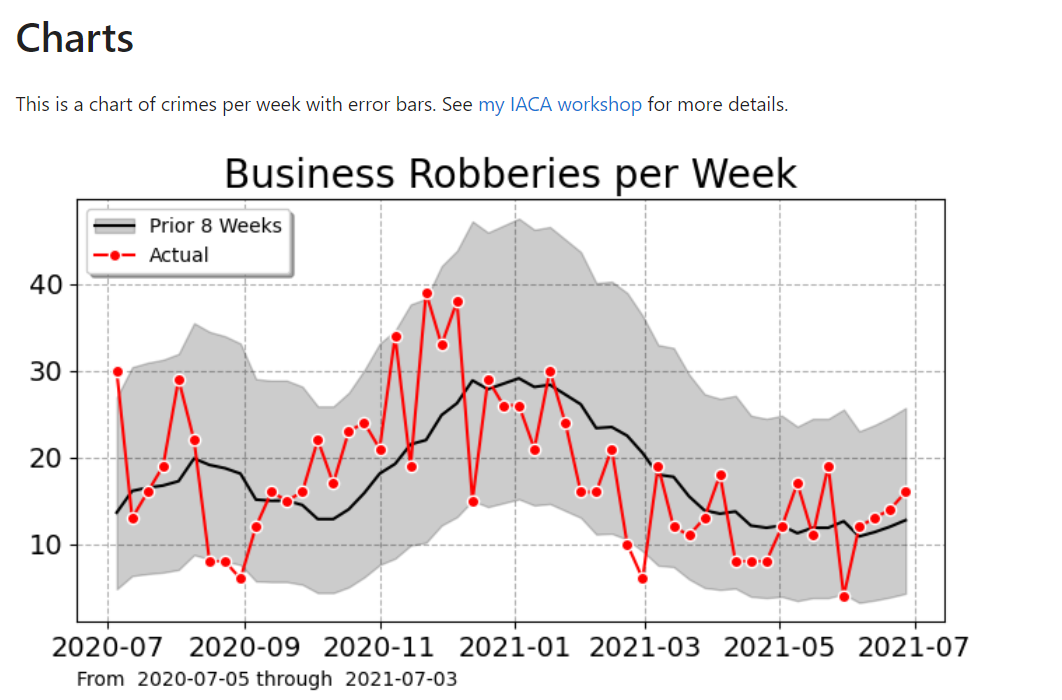So this is an uber nerd post – I hope it has general lessons though. One is that if you need to estimate `Y`, and you can write Y as a function of other variables, some that are variable and some that are not, e.g. `Y = f(x1,c)`, then maybe you should just focus on estimating `x1` in this scenario, not model Y directly.

In terms of more general statistical modeling of crime trends, I have debated in the past examining more thoroughly seasonal-trend decomposition techniques, but I think the examples I give above are quite sufficient for most analysis (and can be implemented in a spreadsheet).

# My journey submitting to CRAN

So my R package ptools is up on CRAN. CRAN obviously does an important service – I find the issues I had to deal with pedantic – but will detail my struggles here, mostly so others hopefully do not have to deal with the same issues in the future. Long story short I knew going in it can be tough and CRAN did not disappoint.

Initially I submitted the package in early June, which it passed the email verification, but did not receive any email back after that. I falsely presumed it was in manual review. After around a month I sent an email to cran-sysadmin. The CRAN sysadmin promptly sent an email back with the reason it auto-failed – examples took too long – but not sure why I did not receive an auto-message back (so it never got to the manual review stage). When I got auto-fail messages at the equivalent stage in later submissions, it was typically under an hour to get that stage auto-fail message back.

So then I went to fixing the examples that took too long (which on my personal machine all run in under 5 seconds, I have a windows \$400 low end “gaming” desktop, with an extra \$100 in RAM, so I am not running some supercomputer here as background). Running devtools `check()` is not the same as running `R CMD check --as-cran path\package.tar.gz`, but maybe `check_built()` is, I am not sure. So first note to self just use the typical command line tools and don’t be lazy with devtools.

Initially I commented out sections of the examples that I knew took too long. Upon manual review though, was told don’t do that and to wrap too long of examples in `donttest{}`. Stochastic changes in run times even made me fail a few times at this – some examples passed the time check in some runs but failed in others. Some examples that run pretty much instantly on my machine failed in under 10 seconds for windows builds on CRAN’s checks. (My examples use plots on occasion, and it may be `spplot` was the offender, as well as some of my functions that are not fast and use loops internally.) I have no advice here than to just always wrap plot functions in `donttest{}`, as well as anything too complicated for an abacus. There is no reliable way (that I can figure) to know examples that are very fast on my machine will take 10+ seconds on CRAN’s checks.

But doing all of these runs resulted in additional Notes in the description about spelling errors. At first it was last names in citations (Wheeler and Ratcliffe). So I took those citations out to prevent the Note. Later in manual review I was asked to put them back in. Occasionally a DOI check would fail as well, although it is the correct DOI.

One of the things that is confusing to me – some of the Note’s cause automatic failures (examples too long) and others do not (spelling errors, DOI check). The end result messages to me are the same though (or at least I don’t know how to parse a “this is important” Note vs a “whatever not a big deal” Note). The irony of this back and forth related to these spelling/DOI notes in the description is that the description went through changes only to get back to what is was originally.

So at this point (somewhere around 10+ submission attempts), 7/16, it finally gets past the auto/human checks to the point it is uploaded to CRAN. Finished right – false! I then get an automated email from Brian Ripley/CRAN later that night saying it is up, but will be removed on 8/8 because `Namespace in Imports field not imported from: 'maptools'`.

One function had `requireNamespace("maptools")` to use the conversion functions in maptools to go between sp/spatspat objects. This caused that “final” note about maptools not being loaded. To fix this, I ended up just removing maptools dependency altogether, as using unexported functions, e.g. `maptools:::func` causes a note when I run `R CMD check` locally (so presume it will auto-fail). There is probably a smarter/more appropriate way to use imports – I default though to doing something I hope will pass the CRAN checks though.

I am not sure why this namespace is deal breaker at this stage (after already on CRAN) and not earlier stages. Again this is another Note, not a warning/error. But sufficient to get CRAN to remove my package in a few weeks if I don’t fix. This email does not have the option “send email if a false positive”.

When resubmitting after doing my fixes, I then got a new error for the same package version (because it technically is on CRAN at this point), so I guess I needed to increment to 1.0.1 and not fix the 1.0.0 package at this point. Also now the DOI issue in the description causes a “warning”. So I am not sure if this update failed because of package version (which doesn’t say note or warning in the auto-fail email) or because of DOI failure (which again is now a warning, not a Note).

Why sometimes a DOI failure is a warning and other times it is a note I do not know. At some later stage I just take this offending DOI out (against the prior manual review), as it can cause auto-failures (all cites are in the examples/docs as well).

OK, so package version incremented and namespace error fixed. Now in manual review for the 1.0.1 version, get a note back to fix my errors – one of my tests fails on noLD/M1Mac (what is noLD you may ask? It is “no long doubles”). These technically failed on prior as well, but I thought I just needed to pass 2+ OS’s to get on CRAN. I send an email to Uwe Ligges at this point (as he sent an email about errors in prior 1.0.0 versions at well) to get clarity about what exactly they care about (since the reason I started round 2 was because of the Namespace threat, not the test errors on Macs/noLD). Uwe responds very fast they care about my test that fails, not the DOI/namespace junk.

So in some of my exact tests I have checks along the line `ref <- c(0.25,0.58); act <- round(f,2)` where `f` is the results scooped up from my prior function calls. The note rounds the results to the first digit, e.g. `0.2 0.5` in the failure (I suspect this is some behavior for `testhat` in terms of what is printed to the console for the error, but I don’t know how exactly to fix the function calls so no doubles will work). I just admit defeat and comment out the part of this test function that I think is causing the failure, any solution I am not personally going to be able to test in my setup to see if it works. Caveat Emptor, be aware my exact test power calculation functions are not so good if you are on a machine that can’t have long doubles (or M1 Mac’s I guess, I don’t fricken know).

OK, so that one test fixed, upon resubmission (the following day) I get a new error in my tests (now on Windows) – `Error in sp::CRS(...): PROJ4 argument-value pairs must begin with +`. I have no clue why this is showing an error now, for the first time going on close to 20 submissions over the past month and a half.

The projection string I pass definitely has a “+” at the front – I don’t know and subsequent submissions to CRAN even after my attempts to fix (submitting projections with simpler epsg codes) continue to fail now. I give up and just remove that particular test.

Uwe sends an updated email in manual review, asking why I removed the tests and did not fix them (or fix my code). I go into great detail about the new SP error (that I don’t think is my issue), and that I don’t know the root cause of the noLD/Mac error (and I won’t be able to debug before 8/8), that the code has pretty good test coverage (those functions pass the other tests for noLD/Mac, just one), and ask for his grace to upload. He says OK patch is going to CRAN. It has been 24 hours since then, so cannot say for sure I will not get a ‘will be removed’ auto-email.

To be clear these issues back and forth are on me (I am sure the `\donttest{}` note was somewhere in online documentation that I should have known). About the only legit complaint I have in the process is that the “Note” failure carries with it some ambiguity – some notes are deal breakers and others aren’t. I suspect this is because many legacy packages fail these stringent of checks though, so they need to not auto-fail and have some discretion.

The noLD errors make me question reality itself – does `0.25 = 0.2` according to M1 Mac’s? Have I been living a lie my whole life? Do I really know my code works? I will eventually need to spin up a Docker image and try to replicate the noLD environment to know what is going on with that one exact test power function.

For the projection errors, I haven’t travelled much recently – does Long Island still exist? Is the earth no longer an ellipsoid? At our core are we just binary bits flipping the neural networks of our brain – am I no better than the machine?

There is an irony here that people with better test code coverage are more likely to fail the auto-checks (although those packages are also more likely to be correct!). It is intended and reasonable behavior from CRAN, but it puts a very large burden on the developer (it is not easy to debug noLD behavior on your own, and M1 Mac’s are effectively impossible unless you wish to pony up the cash for one).

CRAN’s model is much different than python’s PyPI, in that I could submit something to PyPI that won’t install at all, or will install but cause instant errors when running `import mypackage`. CRANs approach is more thorough, but as I attest to above is quite a bit on the pedantic side (there are no “functional” changes to my code in the last month I went through the back and forth).

The main thing I really care about in a package repository is that it does not have malicious code that does suspicious `os` calls and/or sends suspicious things over the internet. It is on me to verify the integrity of the code in the end (even if the examples work it doesn’t mean the code is correct, I have come across a few packages on R that have functions that are obviously wrong/misleading). This isn’t an open vs closed source thing – you need to verify/sanity check some things work as expected on your own no matter what.

So I am on the fence whether CRAN’s excessive checking is “worth it” or not. Ultimately since you can do:

``````library(devtools)
install_github("apwheele/ptools")``````

Maybe it does not matter in the end. And you can peruse the github actions to see the current state of whether it runs on different operating systems and avoid CRAN altogether.

# Staggered Treatment Effect DiD count models

So I have been dealing with various staggered treatments for difference-in-difference (DiD) designs for crime data analysis on how interventions reduce crime. I’ve written about in the past mine and Jerry’s WDD estimator (Wheeler & Ratcliffe, 2018), as well as David Wilson’s ORR estimator (Wilson, 2022).

There has been quite a bit of work in econometrics recently describing how the traditional way to apply this design to staggered treatments using two-way fixed effects can be misleading, see Baker et al. (2022) for human readable overview.

The main idea is that in the scenario where you have treatment heterogeneity (TH from here on) (either over time or over units), the two-way fixed effects estimator is a weird average that can misbehave. Here are just some notes of mine though on fitting the fully saturated model, and using post-hoc contrasts (in R) to look at that TH as well as to estimate more reasonable average treatment effects.

So first, we can trick R to use `glm` to get my WDD estimator (or of course Wilson’s ORR estimator) for the DiD effect with count data. Here is a simple example from my prior blog post:

``````# R code for DiD model of count data
count <- c(50,30,60,55)
post <- c(0,1,0,1)
treat <- c(1,1,0,0)

df <- data.frame(count,post,treat)

# Wilson ORR estimate
m1 <- glm(count ~ post + treat + post*treat,data=df,family="poisson")
summary(m1)``````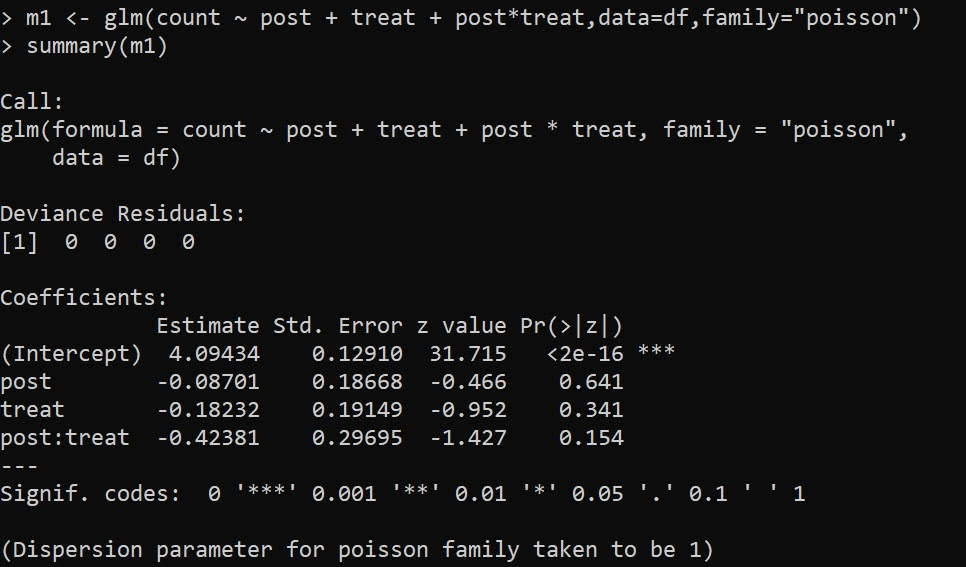And here is the WDD estimate using `glm` passing in `family=poisson(link="identity")`:

``````m2 <- glm(count ~ post + treat + post*treat,data=df,
summary(m2)``````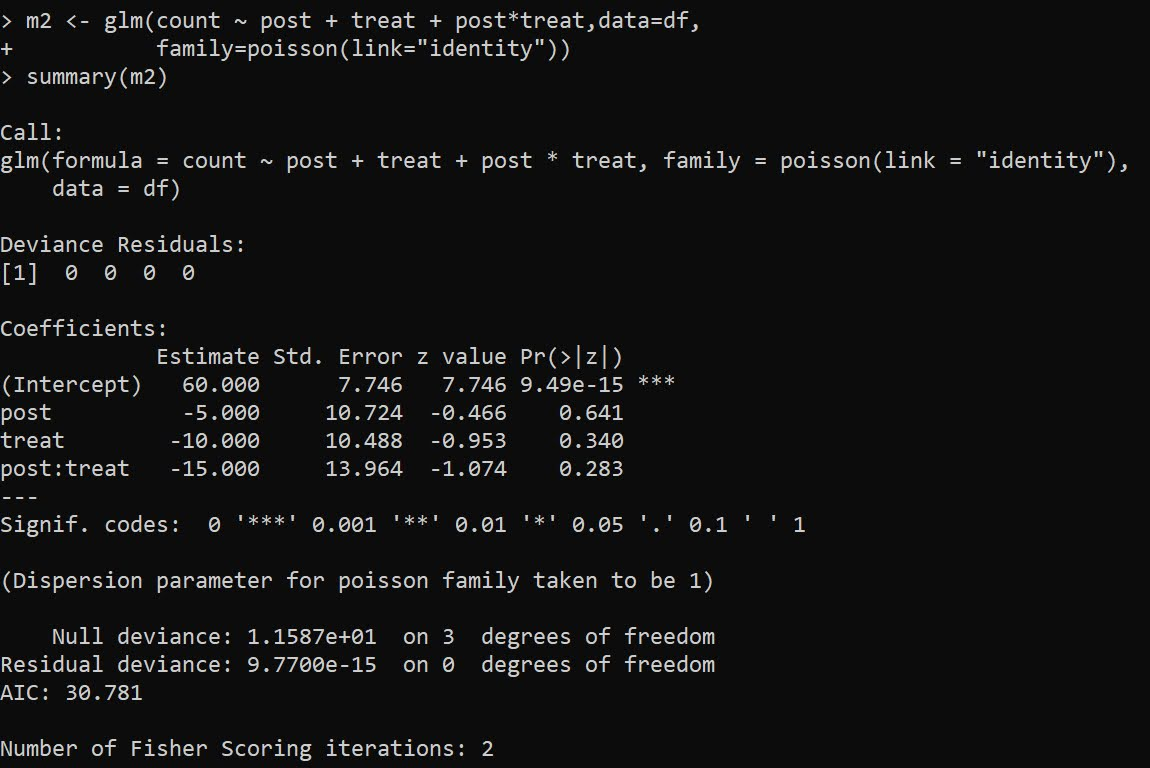And we can see this is the same as my WDD in the ptools package:

``````library(ptools) # via https://github.com/apwheele/ptools
wdd(c(60,55),c(50,30))``````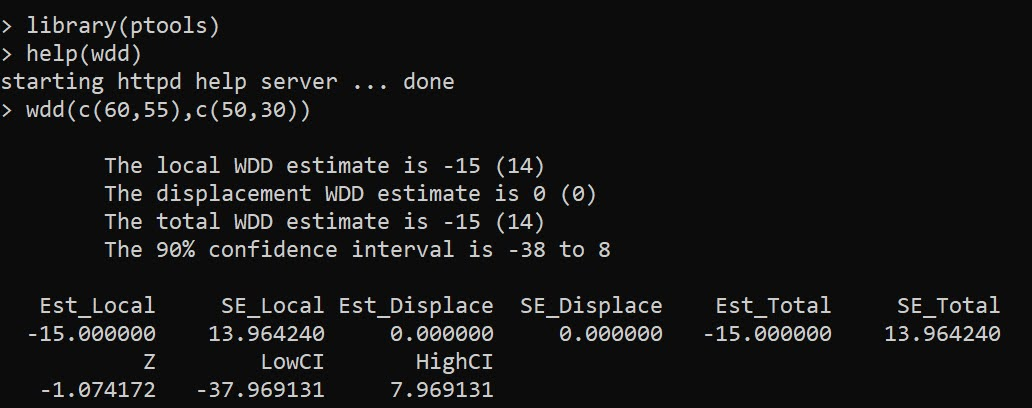Using glm will be more convenient than me scrubbing up all the correct weights, as I’ve done in the past examples (such as temporal weights and different area sizes). It is probably the case you can use different offsets in regression to accomplish similar things, but for this post just focusing on extending the WDD to varying treatment timing.

## Varying Treatment Effects

So the above scenario is a simple pre/post with only one treated unit. But imagine we have two treated units and three time periods. This is very common in real life data where you roll out some intervention to more and more areas over time.

So imagine we have a set of crime data, `G1` is rolled out first, so the treatment is turned on for periods `One & Two`, `G2` is rolled out later, and so the treatment is only turned on for period `Two`.

``````Period    Control     G1     G2
Base          50      70     40
One           60      70     50
Two           70      80     50``````

I have intentionally created this example so the average treatment effect per period per unit is 10 crimes. So no TH. Here is the R code to show off the typical default two-way fixed effects model, where we just have a dummy variable for `unit+timeperiods` that are treated.

``````# Examples with Staggered Treatments
Period    Control     G1     G2
Base          50      70     40
One           60      70     50
Two           70      80     50
")

# reshape wide to long
nvars <- c("Control","G1","G2")
dfl <- reshape(df,direction="long",
idvar="Period",
varying=list(nvars),
timevar="Unit")

dfl\$Unit <- as.factor(dfl\$Unit)
names(dfl) <- 'Crimes'

# How to set up design matrix appropriately?
dfl\$PostTreat <- c(0,0,0,0,1,1,0,0,1)

m1 <- glm(Crimes ~ PostTreat + Unit + Period,
data=dfl)

summary(m1) # TWFE, correct point estimate``````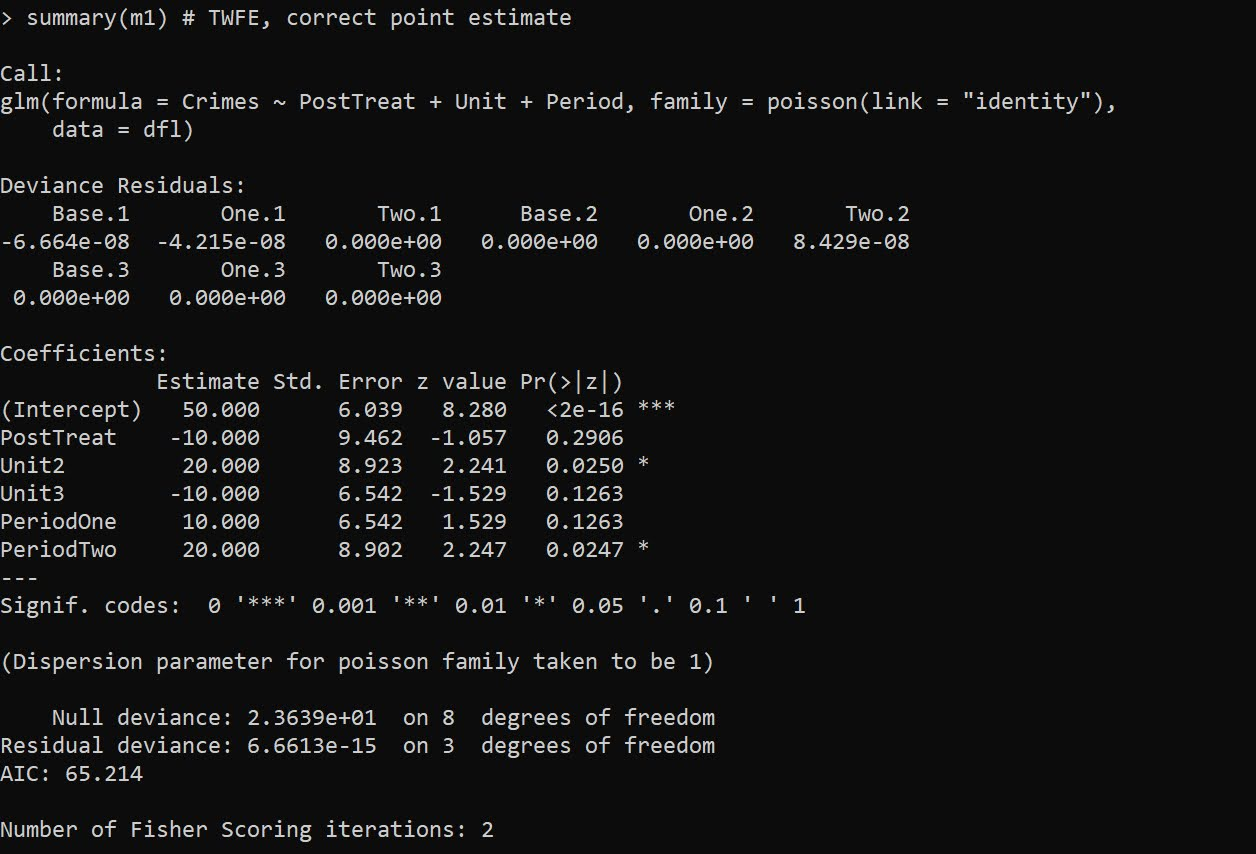The `PostTreat` variable is the one we are interested in, and we can see that we have the correct `-10` estimate as we expected.

OK, so lets create some treatment heterogeneity, here now `G1` has no effects, and only `G2` treatment works.

``````dfl[dfl\$Unit == 2,'Crimes'] <- c(70,80,90)

m2 <- glm(Crimes ~ PostTreat + Unit + Period,
data=dfl)

summary(m2) # TWFE, estimate -5.29, what?``````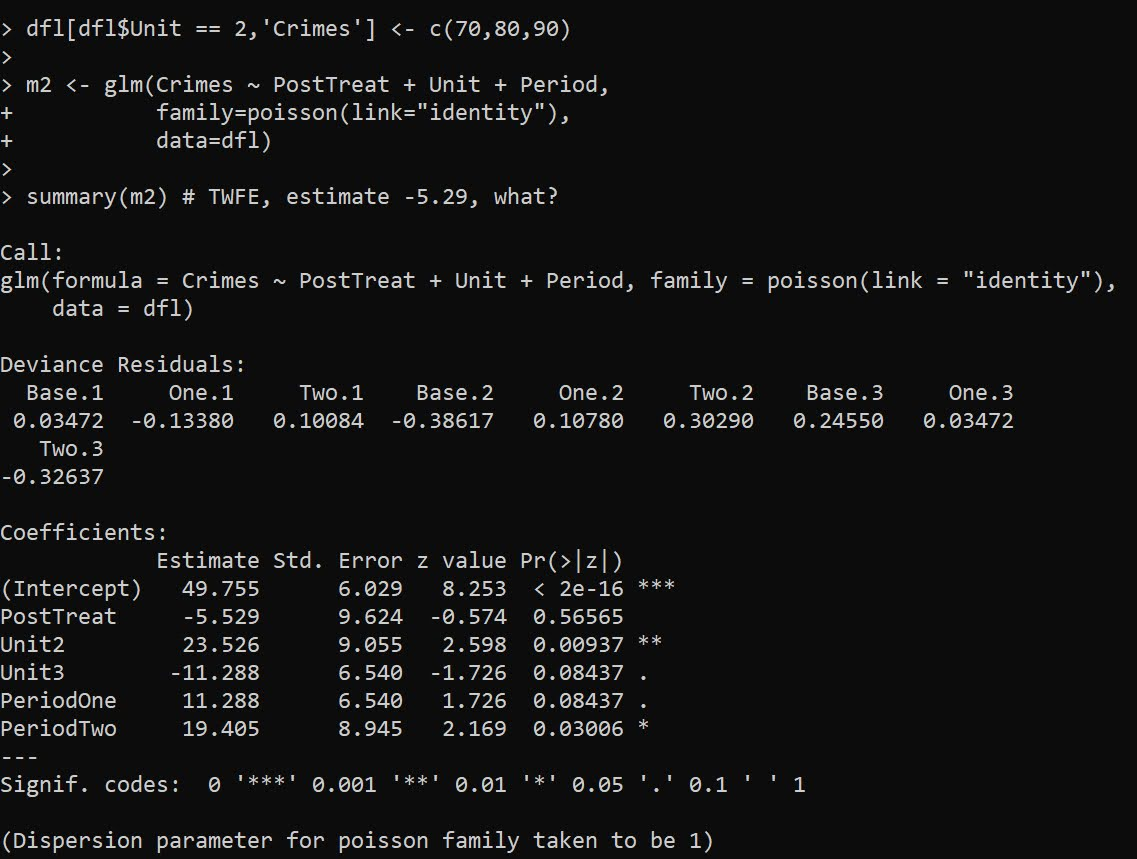So you may naively think that this should be something like -5 (average effect of G1 + G2), or -3.33 (G1 gets a higher weight since it is turned on for the 2 periods, whereas G2 is only turned on for 1). But nope rope, we get -5.529.

We can estimate the effects of `G1` and `G2` seperately though in the regression equation:

``````# Lets seperate out the two units effects
dfl\$pt1 <- 1*(dfl\$Unit == 2)*dfl\$PostTreat
dfl\$pt2 <- 1*(dfl\$Unit == 3)*dfl\$PostTreat

m3 <- glm(Crimes ~ pt1 + pt2 + Unit + Period,
data=dfl)

summary(m3) # Now we get the correct estimates``````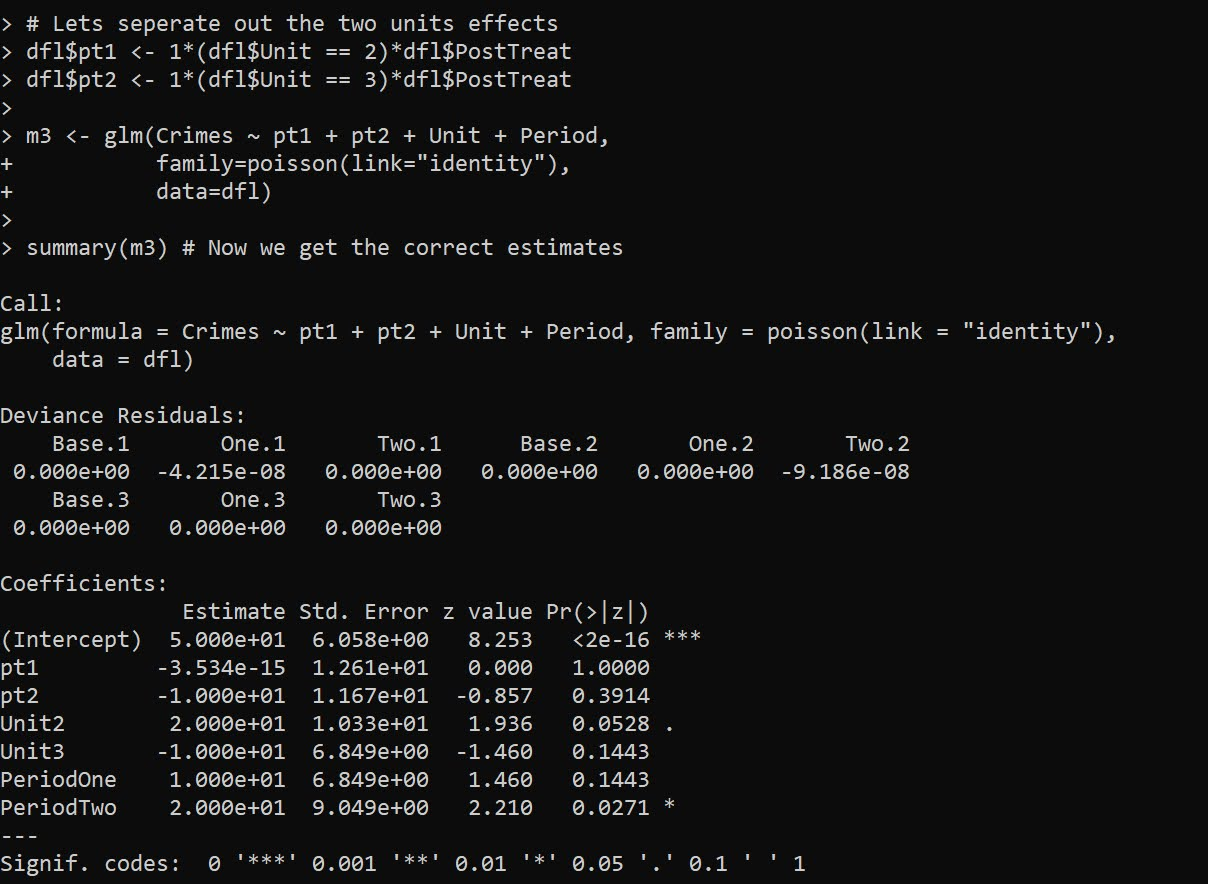And now we can see that as expected, the effect for `G2` is the `pt2` coefficient, which is -10. And the effect for `G1`, the `pt1` coefficient, is only floating point error different than 0.

To then get a cumulative crime reduction effect for all of the areas, we can use the `multcomp` library and the `glht` function and construct the correct contrast matrix. Here the `G1` effect gets turned on for 2 periods, and the `G2` effect is only turned on for 1 period.

``````library(multcomp)
cont <- matrix(c(0,2,1,0,0,0,0),1)
cumtreat <- glht(m3,cont) # correct cumulative
summary(cumtreat)``````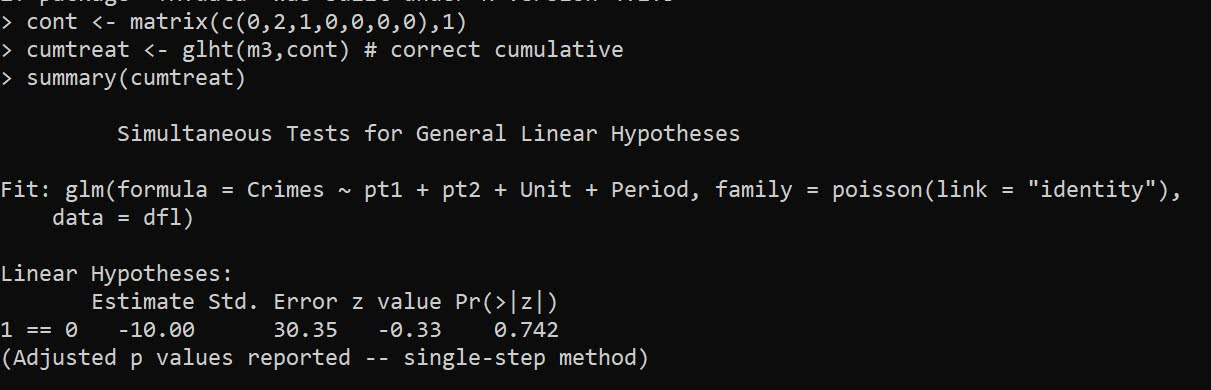And if we want an ‘average treatment effect per unit and per period’, we just change the weights in the contrast matrix:

``````atreat <- glht(m3,cont/3) # correct average over 3 periods
summary(atreat)``````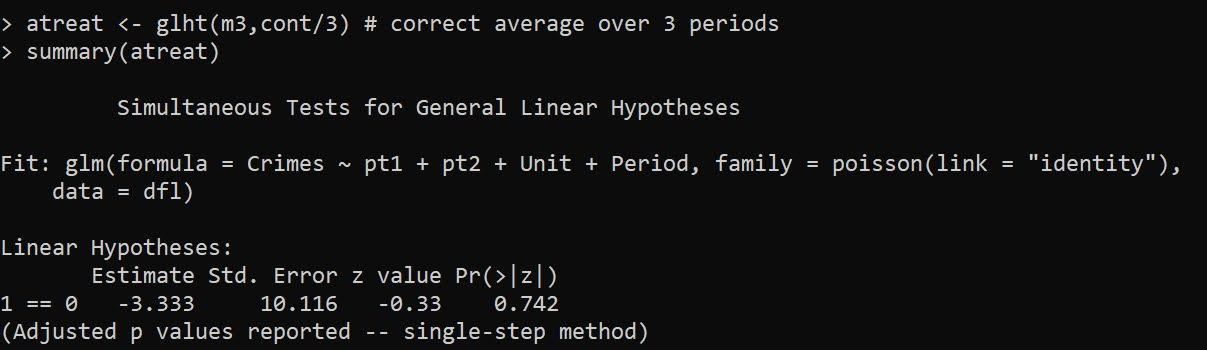And this gets us our -3.33 that is a more reasonable average treatment effect. Although you would almost surely just focus on that the G2 area intervention worked and the G1 area did not.

You can also fit this model alittle bit easier using R’s style formula instead of rolling your own dummy variables via the formula `Crimes ~ PostTreat:Unit + Unit + Period`: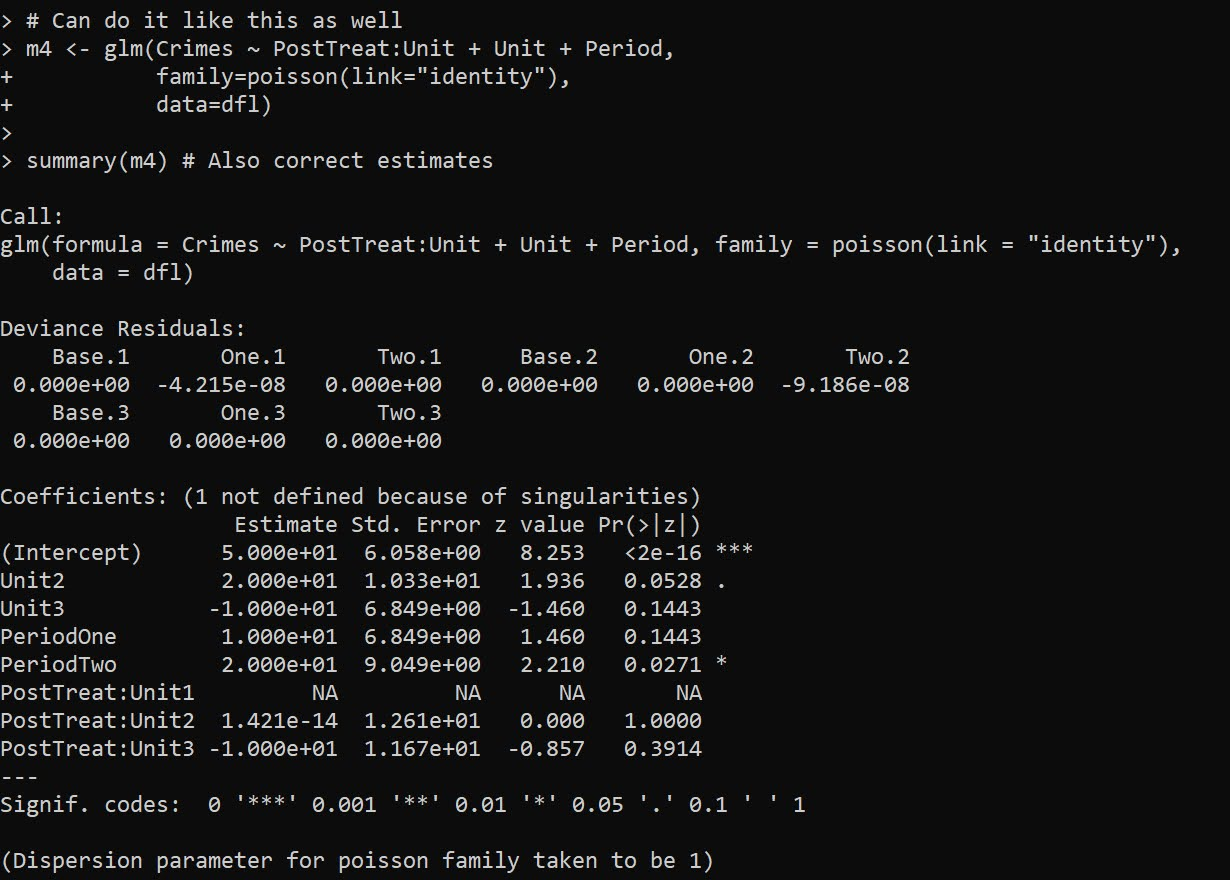But, `glht` does not like it when you have dropped levels in these interactions, so I don’t do this approach directly later on, but construct the model matrix and drop non-varying columns.

Next lets redo the data again, and now have time varying treatments. Now only period 2 is effective, but it is effective across both the G1 and G2 locations. Here is how I construct the model matrix, and what the resulting sets of dummy variables looks like:

``````# Time Varying Effects
# only period 2 has an effect

dfl[dfl\$Unit == 2,'Crimes'] <- c(70,80,80)

# Some bookkeeping to make the correct model matrix
mm <- as.data.frame(model.matrix(~ -1 + PostTreat:Period + Unit + Period, dfl))
mm <- mm[,names(mm)[colSums(mm) > 0]] # dropping zero columns
names(mm) <- gsub(":","_",names(mm))  # replacing colon
mm\$Crimes <- dfl\$Crimes
print(mm)``````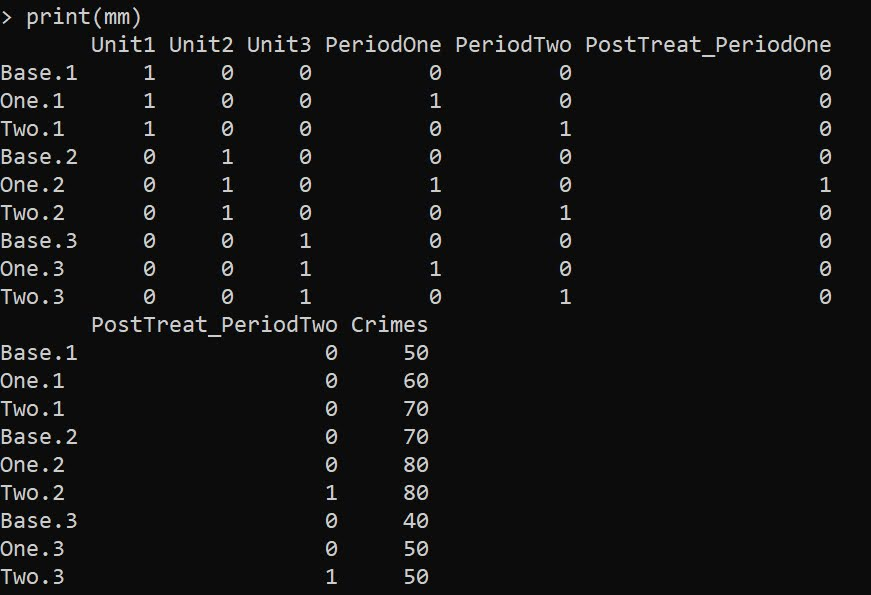Now we can go ahead and fit the model without the intercept.

``````# Now can fit the model
m6 <- glm(Crimes ~ . -1,
data=mm)

summary(m6)``````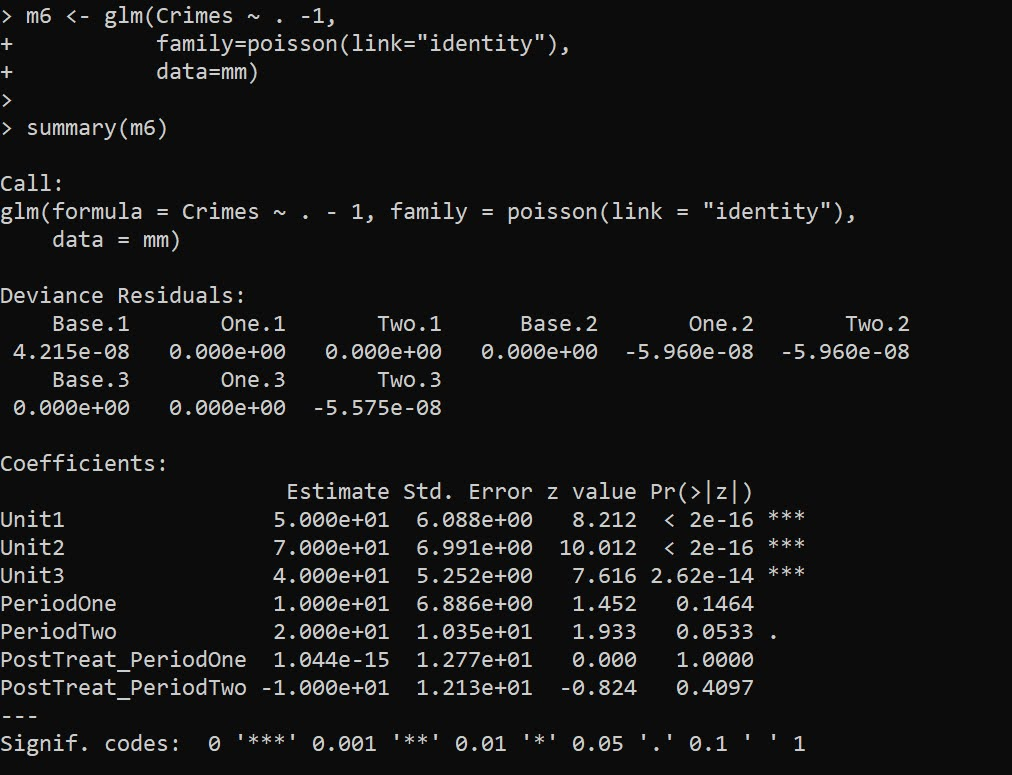And you can see we estimate the correct effects here, `PostTreat_PeriodOne` has a zero estimate, and `PostTreat_PeriodTwo` has a -10 estimate. And now our cumulative crimes reduced estimate -20

``````cumtreat2 <- glht(m6,"1*PostTreat_PeriodOne + 2*PostTreat_PeriodTwo=0")
summary(cumtreat2)``````And if we did the average, it would be `-6.66`.

Now for the finale – we can estimate the saturated model with time-and-unit varying treatment effects. Here is what the design matrix looks like, just a bunch of columns with a single 1 turned on:

``````# Now for the whole shebang, unit and period effects
mm2 <- as.data.frame(model.matrix(~ -1 + Unit:PostTreat:Period + Unit + Period, dfl))
mm2 <- mm2[,names(mm2)[colSums(mm2) > 0]] # dropping zero columns
names(mm2) <- gsub(":","_",names(mm2))  # replacing colon
mm2\$Crimes <- dfl\$Crimes
print(mm2)``````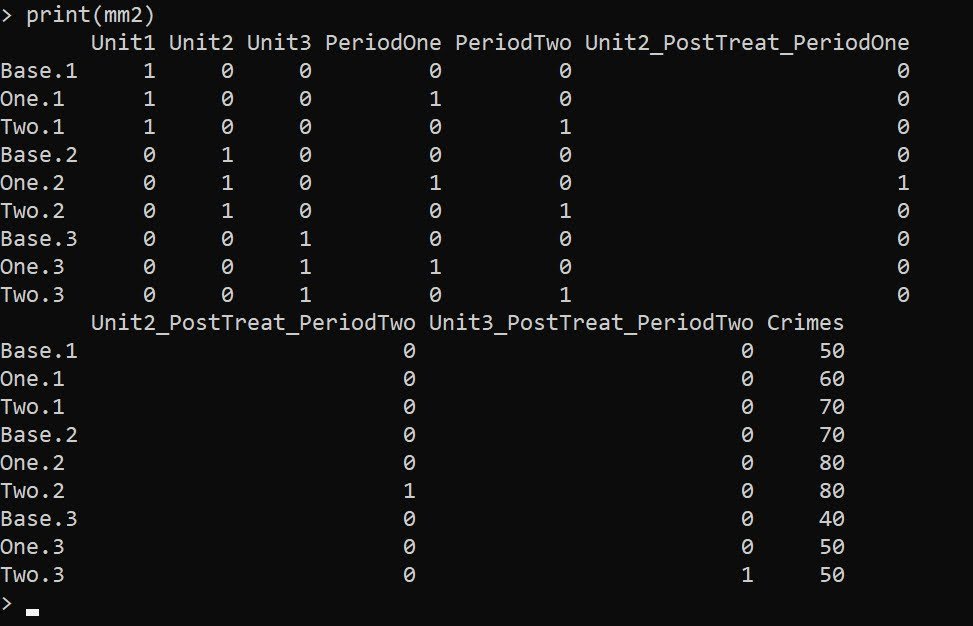And then we can fit the model the same way:

``````m7 <- glm(Crimes ~ . -1,
data=mm2)

summary(m7) # Now we get the correct estimates``````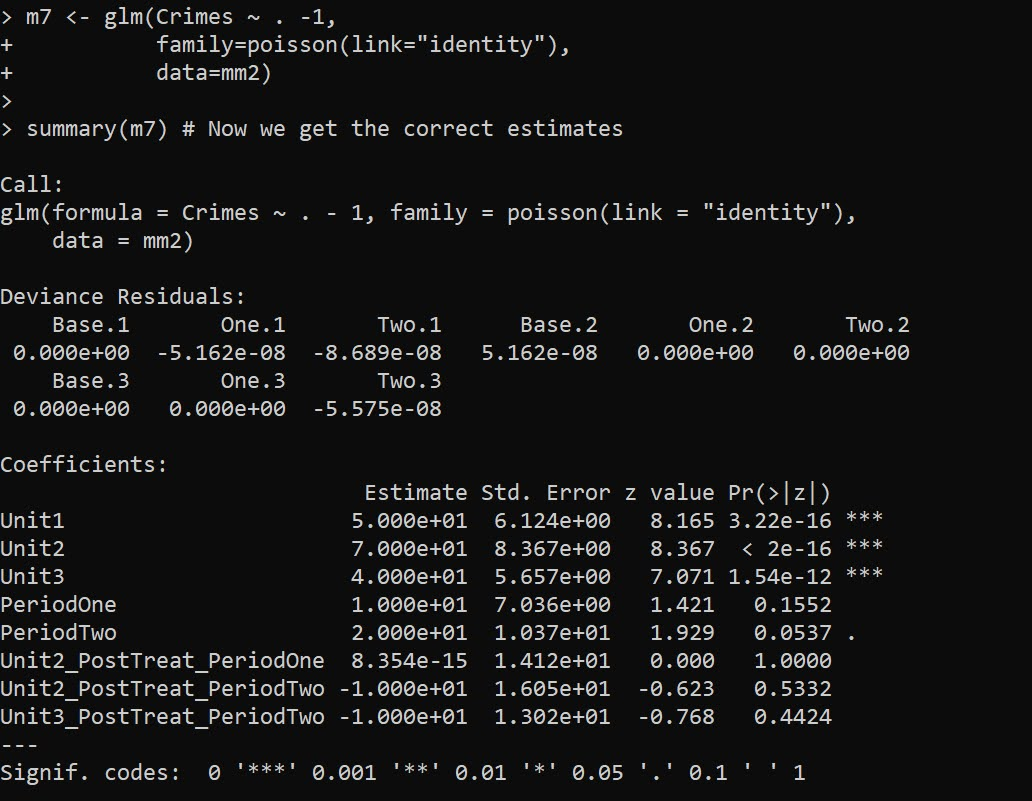And you can see our -10 estimate for `Unit2_PostTreat_PeriodTwo` and `Unit3_PostTreat_PeriodTwo` as expected. You can probably figure out how to get the cumulative or the average treatment effects at this point:

``````tstr <- "Unit2_PostTreat_PeriodOne + Unit2_PostTreat_PeriodTwo + Unit3_PostTreat_PeriodTwo = 0"
cumtreat3 <- glht(m7,tstr)
summary(cumtreat3)``````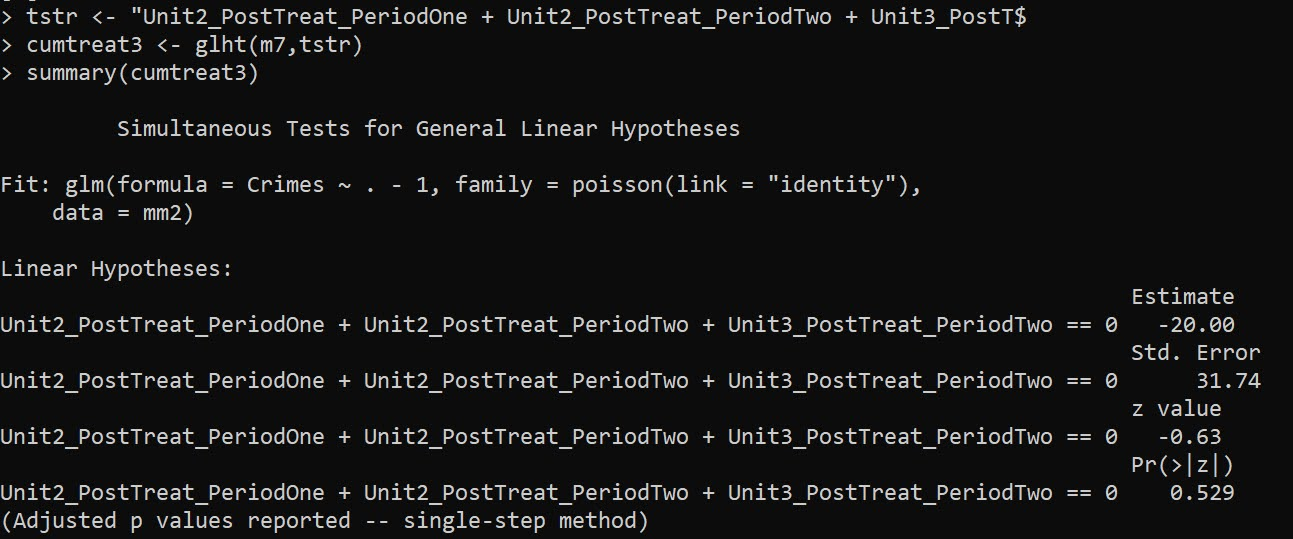We can also use this same framework to get a unit and time varying estimate for Wilson’s ORR estimator, just using `family=poisson` with its default log link function: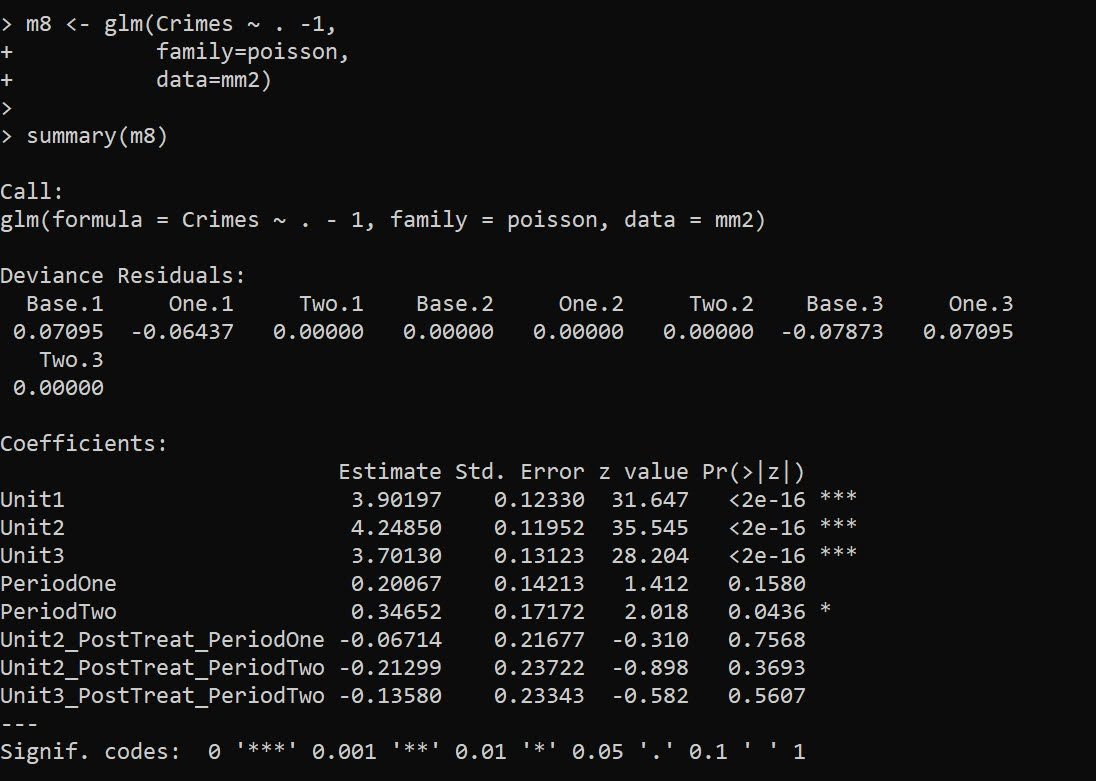``````m8 <- glm(Crimes ~ . -1,
family=poisson,
data=mm2)

summary(m8)``````

It probably does not make sense to do a cumulative treatment effect in this framework, but I think an average is OK:

``````avtreatorr <- glht(m8,
"1/3*Unit2_PostTreat_PeriodOne + 1/3*Unit2_PostTreat_PeriodTwo + 1/3*Unit3_PostTreat_PeriodTwo = 0")
summary(avtreatorr)``````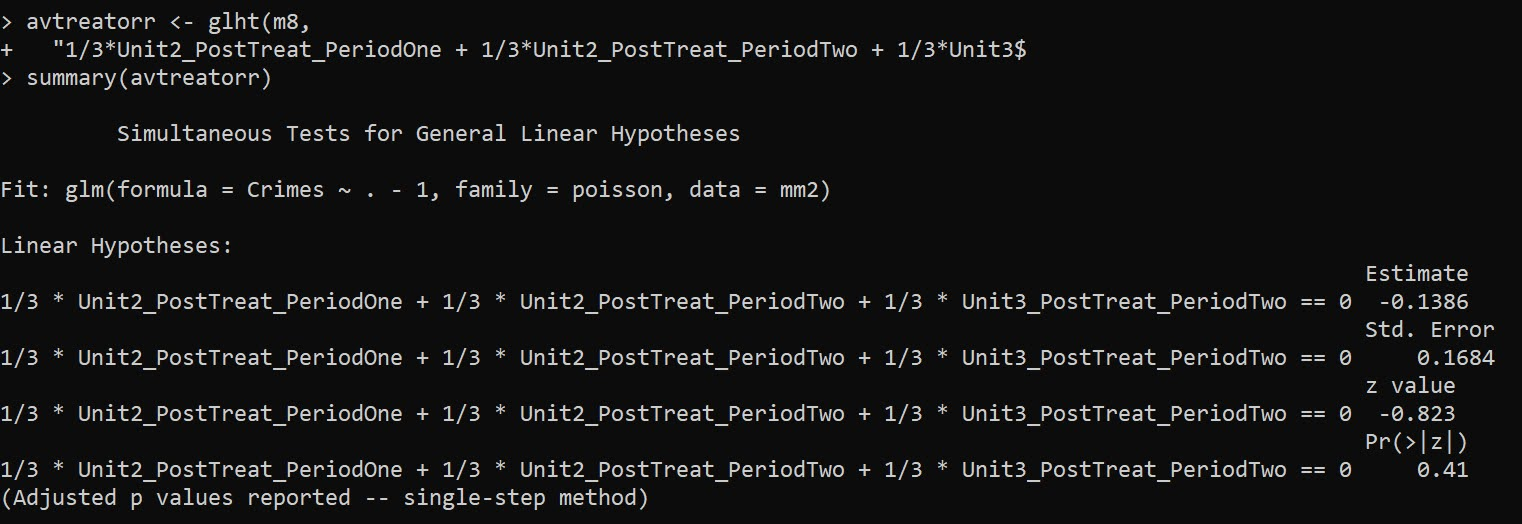So the average linear coefficient is -0.1386, and if we exponentiate that we have an IRR of `0.87`, so on average when a treatment occurred in this data a 13% reduction. (But beware, I intentionally created this data so the parallel trends for the DiD analysis were linear, not logarithmic).

Note if you are wondering about robust estimators, Wilson suggests using quasipoisson, e.g. `glm(Crimes ~ . -1,family="quasipoisson",data=mm2)`, which works just fine for this data. The quasipoisson or other robust estimators though return 0 standard errors for the saturated `family=poisson(link="identity")` or `family=quasipoisson(link="identity")`.

E.g. doing

``````library(sandwich)
cumtreat_rob <- glht(m7,tstr,vcov=vcovHC,type="HC0")
summary(cumtreat_rob)``````

Or just looking at robust coefficients in general:

``````library(lmtest)
coeftest(m7,vcov=vcovHC,type="HC0")``````

Returns 0 standard errors. I am thinking with the saturated model and my WDD estimate, you get the issue with robust standard errors described in Mostly Harmless Econometrics (Angrist & Pischke, 2008), that they misbehave in small samples. So I am a bit hesitant to suggest them without more work to establish they behave the way they should in smaller samples.

• Angrist, J.D., & Pischke, J.S. (2008). Mostly Harmless Econometrics. Princeton University Press.
• Baker, A.C., Larcker, D.F., & Wang, C.C. (2022). How much should we trust staggered difference-in-differences estimates? Journal of Financial Economics, 144(2), 370-395.
• Wheeler, A.P., & Ratcliffe, J.H. (2018). A simple weighted displacement difference test to evaluate place based crime interventions. Crime Science, 7(1), 1-9.
• Wilson, D.B. (2022). The relative incident rate ratio effect size for count-based impact evaluations: When an odds ratio is not an odds ratio. Journal of Quantitative Criminology, 38(2), 323-341.

# An update on the WaPo Officer Involved Shooting Stats

Marisa Iati interviewed me for a few clips in a recent update of the WaPo data on officer involved fatal police shootings. I’ve written in the past the data are very consistent with a Poisson process, and this continues to be true.

So first thing Marisa said was that shootings in 2021 are at 1055 (up from 1021 in 2020). Is this a significant increase? I said no off the cuff – I knew the average over the time period WaPo has been collecting data is around 1000 fatal shootings per year, so given a Poisson distribution mean=variance, we know the standard deviation of the series is close to `sqrt(1000)`, which approximately equals 60. So anything 1000 plus/minus 60 (i.e. 940-1060) is within the typical range you would expect.

In every interview I do, I struggle to describe frequentist concepts to journalists (and this is no different). This is not a critique of Marisa, this paragraph is certainly not how I would write it down on paper, but likely was the jumble that came out of my mouth when I talked to her over the phone:

Despite setting a record, experts said the 2021 total was within expected bounds. Police have fatally shot roughly 1,000 people in each of the past seven years, ranging from 958 in 2016 to last year’s high. Mathematicians say this stability may be explained by Poisson’s random variable, a principle of probability theory that holds that the number of independent, uncommon events in a large population will remain fairly stagnant absent major societal changes.

So this sort of mixes up two concepts. One, the distribution of fatal officer shootings (a random variable) can be very well approximated via a Poisson process. Which I will show below still holds true with the newest data. Second, what does this say about potential hypotheses we have about things that we think might influence police behavior? I will come back to this at the end of the post,

# R Analysis at the Daily Level

So my current ptools R package can do a simple analysis to show that this data is very consistent with a Poisson process. First, install the most recent version of the package via devtools, then you can read in the WaPo data directly via the Github URL:

``````library(devtools)
install_github("apwheele/ptools")
library(ptools)

url <- 'https://raw.githubusercontent.com/washingtonpost/data-police-shootings/master/fatal-police-shootings-data.csv'

Looking at the yearly statistics (clipping off events recorded so far in 2022), you can see that they are hypothetically very close to a Poisson distribution with a mean/variance of 1000, although perhaps have a slow upward trend over the years.

``````# Year Stats
oid\$year <- as.integer(substr(oid\$date,1,4))
year_stats <- table(oid\$year)
print(year_stats)
mean(year_stats[1:7]) # average of 1000 per year
var(year_stats[1:7])  # variance just under 1000``````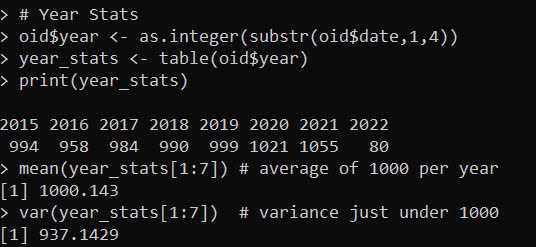We can also look at the distribution at shorter time intervals, here per day. First I aggregat the data to the daily level (including 0 days), second I use my `check_pois` function to get the comparison distributions:

``````#Now aggregating to count per day
oid\$date_val <- as.Date(oid\$date)
date_range <- paste0(seq(as.Date('2015-01-01'),max(oid\$date_val),by='days'))
day_counts <- as.data.frame(table(factor(oid\$date,levels=date_range)))

pfit <- check_pois(day_counts\$Freq, 0, 10, mean(day_counts\$Freq))
print(pfit)``````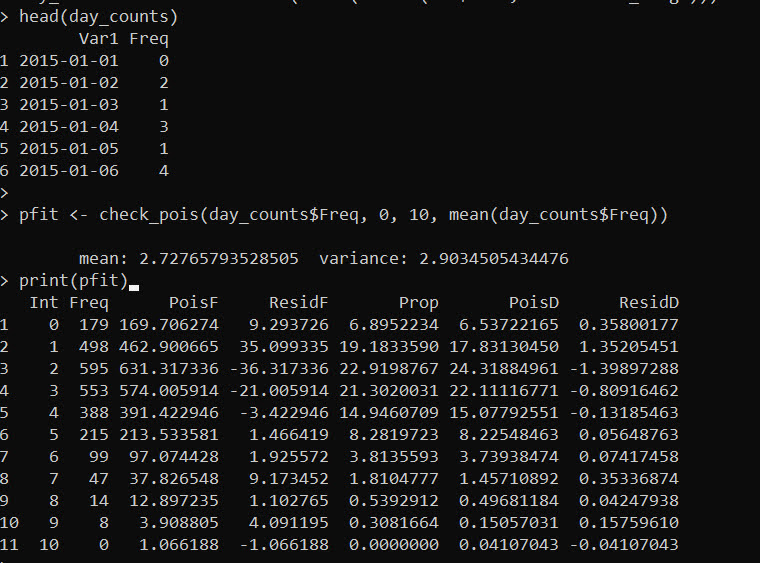The way to read this, for a mean of 2.7 fatal OIS per day (and given this many days), we would expect 169.7 0 fatality days in the sample (`PoisF`), but we actually observed 179 0 fatality days, so a residual of 9.3 in the total count. The trailing rows show the same in percentage terms, so we expect 6.5% of the days in the sample to have 0 fatalities according to the Poisson distribution, and in the actual data we have 6.9%.

You can read the same for the rest of the rows, but it is mostly the same. It is only very slight deviations from the baseline Poisson expected Poisson distribution. This data is the closest I have ever seen to real life, social behavioral data to follow a Poisson process.

For comparison, lets compare to the NYC shootings data I have saved in the ptools package.

``````# Lets check against NYC Shootings
data(nyc_shoot)
date_range <- paste0(seq(as.Date('2006-01-01'),max(nyc_shoot\$OCCUR_DATE),by='days'))
shoot_counts <- as.data.frame(table(factor(nyc_shoot\$OCCUR_DATE,levels=date_range)))

sfit <- check_pois(shoot_counts\$Freq,0,max(shoot_counts\$Freq),mean(shoot_counts\$Freq))
round(sfit,1)``````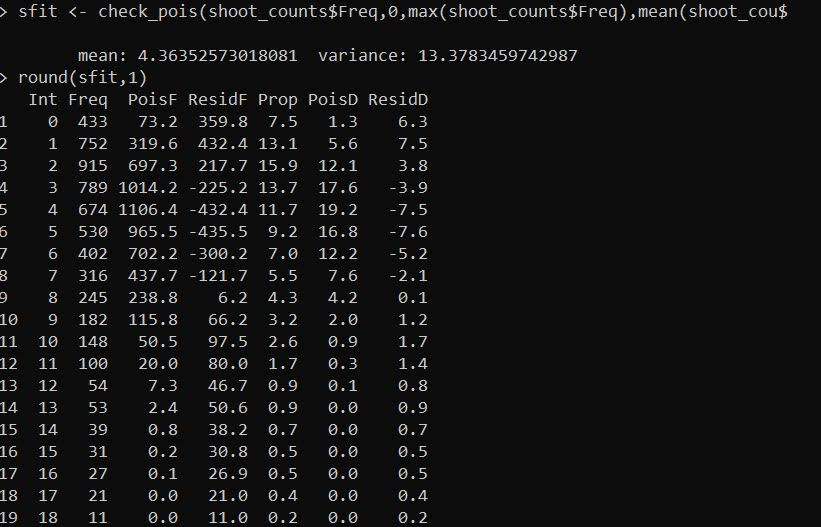This is much more typical of crime data I have analyzed over my career (in that it deviates from a Poisson process by quite a bit). The mean is 4.4 shootings per day, but the variance is over 13. There are many more 0 days than expected (433 observed vs 73 expected). And there are many more high crime shooting days than expected (tail of the distribution even cut off). For example there are 27 days with 18 shootings, whereas a Poisson process would only expect 0.1 days in a sample of this size.

My experience though is that when the data is overdispersed, a negative binomial distribution will fit quite well. (Many people default to a zero-inflated, like Paul Allison I think that is a mistake unless you have a structural reason for the excess zeroes you want to model.)

So here is an example of fitting a negative binomial to the shooting data:

``````# Lets fit a negative binomial and check out
library(fitdistrplus)
fnb <- fitdist(shoot_counts\$Freq,"nbinom")
print(fnb\$estimate)

sfit\$nb <- 100*mapply(dnbinom, x=sfit\$Int, size=fnb\$estimate, mu=fnb\$estimate)
round(sfit[,c('Prop','nb')],1) # Much better overall fit``````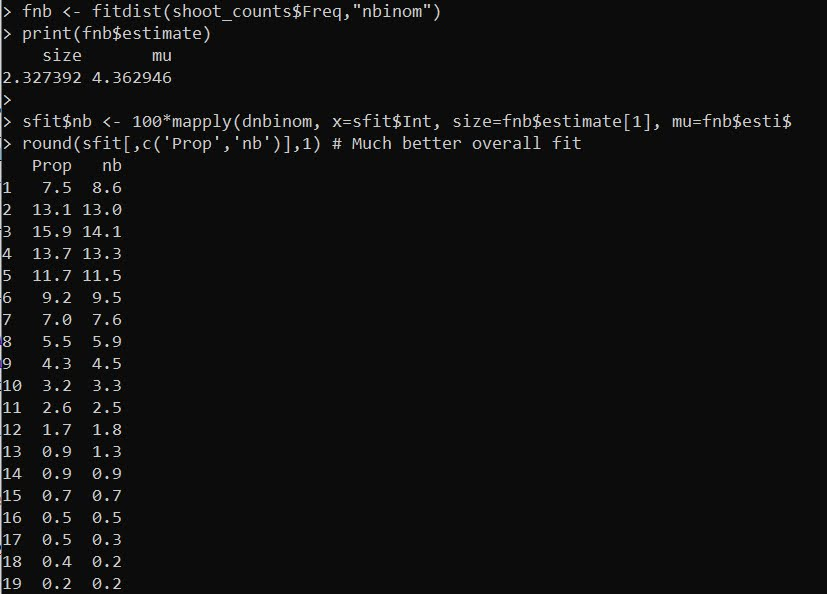And this compares the percentages. So you can see observed 7.5% 0 shooting days, and expected 8.6% according to this negative binomial distribution. Much closer than before. And the tails are fit much closer as well, for example, days with 18 shootings are expected 0.2% of the time, and are observed 0.4% of the time.

# So What Inferences Can We Make?

In social sciences, we are rarely afforded the ability to falsify any particular hypothesis – or in more lay-terms we can’t really ever prove something to be false beyond a reasonable doubt. We can however show whether empirical data is consistent or inconsistent with any particular hypothesis. In terms of Fatal OIS, several ready hypotheses ones may be interested in are Does increased police scrutiny result in fewer OIS?, or Did the recent increase in violence increase OIS?.

While these two processes are certainly plausible, the data collected by WaPo are not consistent with either hypothesis. It is possible both mechanisms are operating at the same time, and so cancel each other out, to result in a very consistent 1000 Fatal OIS per year. A simpler explanation though is that the baseline rate has not changed over time (Occam’s razor).

Again though we are limited in our ability to falsify these particular hypotheses. For example, say there was a very small upward trend, on the order of something like +10 Fatal OIS per year. Given the underlying variance of Poisson variables, even with 7+ years of data it would be very difficult to identify that small of an upward trend. Andrew Gelman likens it to measuring the weight of a feather carried by a Kangaroo jumping on the scale.

So really we could only detect big changes that swing OIS by around 100 events per year I would say offhand. Anything smaller than that is likely very difficult to detect in this data. And so I think it is unlikely any of the recent widespread impacts on policing (BLM, Ferguson, Covid, increased violence rates, whatever) ultimately impacted fatal OIS in any substantive way on that order of magnitude (although they may have had tiny impacts at the margins).

Given that police departments are independent, this suggests the data on fatal OIS are likely independent as well (e.g. one fatal OIS does not cause more fatal OIS, nor the opposite one fatal OIS does not deter more fatal OIS). Because of the independence of police departments, I am not sure there is a real great way to have federal intervention to reduce the number of fatal OIS. I think individual police departments can increase oversight, and maybe state attorney general offices can be in a better place to use data driven approaches to oversee individual departments (like ProPublica did in New Jersey). I wouldn’t bet money though on large deviations from that fatal 1000 OIS anytime soon though.

# Forum posts on Stackoverflow sites

When the initial cross validated stack exchange site (Stackoverflow but for statistics) was formed, I participated a ton. My participation waned though about the time I got a job as a professor. When starting I could skim almost every question, and I learned a ton from that participation. But when the site got more popular that approach was not sustainable. And combined with less time as a professor I just stopped checking entirely.

More recently I have started to simply browse the front page in the morning and only click on questions that look interesting (or I think I could answer reasonably quickly). Most of those answers recently have been Poisson related stuff:

Related I have lost time for the past two weeks, but before that made some good manic progress for my ptools R package. Next step is to make some vignettes for the more complicated spatial feature engineering functions (and maybe either a pre-commit hook to remind me to build the ReadMe, or generate a ReadMe as an artifact using Github actions). The package currently has good documentation, unit tests, and CICD using Github actions.

I also skim the Operations Research site and the Data Science sites. See some recent questions I answered:

The OR site has a really amazing set of people answering questions. I doubt I will ever see a simple enough question fast enough to answer before the multiple guru’s on that site. But I love perusing the answers, similar to when I first started cross validated I have learned quite a bit about formulating linear programming problems.

The data science exchange is at the other end of the spectrum – it is partly due to ill-specified questions, but the level of commentary is very poor (it may in fact be a net negative to the world/internet overall – quite a bit of bad advice). It is lower quality than skimming data science articles on Medium for example (there is some bad stuff on Medium, but overall it is more good than bad that I have seen at least). There is quite a bit of bad data science advice on the internet, and I can see it in the people I am interviewing for DS jobs. This is mainly because quite a bit of DS is statistics, and people seem to rely on copy-pasta solutions without understanding the underlying statistical/decision analysis problems they are solving.

# ptools R package

It has been on my bucket list for a bit, but I wanted to take the time to learn how to construct an R package (same as for a python package). So I crafted a package with only a few functions in it so far, ptools, short for Poisson tools.

These are a handful of functions I have blogged about over the years, including functions for various WDD tests and the variants I have blogged about (weighted harm scores, different time periods, and different area sizes).

Small sample counts in bins (which can be used for Benford’s test), or my original application was checking if a chronic offender had a propensity to commit crimes on certain days of the week.

The Poisson e-test, and a function to check whether a distribution is Poisson distributed and two more Poisson related functions as well.

I think I will add quite a few more functions in the soup before I bother submitting to CRAN. (Installing via devtools via github is quite easy, so I do not feel too bad about that.) If you have functions you think I should add just let me know. (Or just make a pull request and add them yourself!) I also need to work on unit tests, and getting github actions set up. I will probably crunch on this for a bit, and then migrate personal projects back to creating some python libraries for my other work.

I do not use R-studio, but the open book R packages has been immensely helpful. On my windows box I had to bother to add R to my system path, so I can start my R session at the appropriate directory, but besides that very minor hassle it has been quite easy to follow.

I probably have not put in my 10k total hours as a guesstimate to mastery in computer programming. I think maybe closer to 5000, and that is spread out (maybe quite evenly at this point) between python, R, SPSS (and just a little Stata). And I still learn new stuff all the time. Being in an environment where I need to work with more people has really hammered down getting environments right, and making it shareable with other teammates. And part and parcel with that is documenting code in a much more thorough manner than most of the code snippets I leave littered on this blog.

So it probably is worth me posting less, but spending more time making nicer packages to share with everyone.

I do not know really how folks do R programming for making packages. I know a little at this point about creating separate conda environments for python to provide some isolation – is there something equivalent to conda environments for R? Do the `R CMD` checks make this level of isolation unnecessary? Should I just be working on an isolated docker image for all development work? I do not know. I do not have to worry about that at the moment though.

Part of this self learning journey is because I am trying to start a journal aimed at criminologists where you can submit software packages. Similar to the Journal of Open Source Software or the Journal of Statistical Software, etc. For submission to there I want people to have documentation for functions, and really that necessitates having a nice package (whether in R or python or whatever). So I can’t tell people you need to make a package if I don’t do that myself!

The software papers are not a thing yet (I would call it a soft launch at this point), but I have been bugging folks about submitting papers to get a dry run of the process. If you have something you would like to submit, feel free to get in touch and we can get you set up.

# Comparing the WDD vs the Wilson log IRR estimator

So this is maybe my final post on the WDD estimator for the time being (Wheeler & Ratcliffe, 2018). Recently David Wilson had an article in JQC that proposes a different estimator using the same basic information, just pre-post crime counts for treated and control areas (Wilson, 2021). So say we had the table:

``````         Pre   Post
Treated   50     30
Control   60     55``````

So in this scenario, my WDD estimate is -20 in the treated area, and -5 in the control area, so the overall estimate is -20 – -5 = -15.

``30 - 50 - (55 - 60) = -15``

So an estimated reduction of -15 crimes overall. David’s estimator is the logged incident rate ratio (IRR), and so is just like above, except logs all of the values:

``log(30) - log(50) - ( log(55) - log(60) ) = -0.4238142``

This is a logged incident rate adjustment, so most of the time people exponentiate this value, which is `exp(-0.4238142) = 0.6545455`. So this suggests crime is reduced by approximately 35% in the treated area relative to the control area in this hypothetical. Or another way to write it is `(30/50)/(55/60) = 0.6545455`.

So instead of a linear estimate of the total numbers of crimes reduced, this is an estimate of the overall rate reduction. So this begs the question when would you prefer my WDD vs the IRR? I will try to answer that below – in short I think David’s estimator makes sense for meta-analyses (as I have said before in reference to the work in Braga & Weisburd, 2020). But for an individual agency doing an experimental evaluation I much prefer my estimator. The skinny of this logic is that we only really care about the overall crime reduction estimate from a cost-benefit analysis perspective. Backing out this total crime reduction count estimate from David’s IRR estimate can result in some funny business for an individual study.

# Identifying Assumptions

So there are really two different assumptions my WDD estimator and David’s IRR estimator make. To generate a standard error estimate around the point estimate for either estimator, both require the data are Poisson distributed. So that makes no difference between the two. The assumption that really distinguishes between the WDD and the IRR estimate is the parallel trends assumption. The WDD assumes parallel trends are on the linear scale, whereas the IRR assumes parallel trends are on the ratio scale.

What exactly does this mean? Imagine we have a treated and control area, but look at the crime trends per time period before the treatment occurred. This set of areas has a set of parallel trends on the linear scale:

``````Time Treated Control
0     50      60
1     40      50
2     45      55
3     50      60``````

When the treated area goes down by 10 crimes, the control area goes down by 10 crimes. That is a parallel on the linear scale. Whereas this scenario is parallel on the ratio scale:

``````Time Treated Control
0     50      60
1     40      48
2     45      54
3     50      60``````

When crime goes down by 20% in the treated area, it goes down by 20% in the control area.

So while this gives a potential way to say you should use the WDD (parallel on the linear scale), or the IRR (parallel on the ratio scale), in practice it is not so simple. For one thing, if you only has the pre/post counts of crime, you cannot distinguish between these two scenarios. You can only tell in the case you have historical data to examine.

For a second part of this, you typically can choose your own control area (see for example the synthetic control estimator). So in most scenarios you could choose a control area to obey the linear or the ratio parallel trends assumption if you wanted to. However it may be in many scenarios there is a natural/easy control area, and you may see what is a better fit in that case for linear/ratio.

A final wee bit of a perverse aspect about this I will mention – pretend we have a treated/control area have approximately the same baseline crime counts/rates:

``````Time Treated Control
0      30     30
1      25     25
2      20     20
3      25     25``````

You actually cannot tell in this scenario whether the parallel trends are on the linear scale for my WDD or the ratio scale for the IRR estimate. They are consistent with either! In practice I think in many cases it will be like this – with noisy data, if you choose a control area that has approximately the same baseline crime counts, it will be quite hard to tell whether the linear parallel trends makes more sense or the ratio parallel trends makes more sense.

There are situations where the linear changes do not make sense, but they tend to be scenarios such as the control area has very little crime (so cannot go below 0 to match larger ups/downs in the treated area). So in that case sure the IRR is plausible and the WDD is not, but those are cases where the control area itself is quite questionable. Also note the IRR is not defined for any cells with 0 crimes – but again it is not good for either of our estimators in that case (although mine won’t fail to spit out a number, the power is so low the number it spits out won’t be worth much).

# Bias/Coverage

So I have adapted the same simulation code I used in prior studies/blog posts to evaluate the null distribution and the coverage of David’s IRR estimator. I partly did not pursue it initially back when me and Jerry were discussing this idea, because I thought it would be biased. Generalized linear models are based on maximum likelihood estimators, which are only asymptotically valid. In short it appears I was wrong here and David’s IRR estimator is fine even with just four observations, at least for the handful of scenarios I have tried it (have not looked at very tiny counts of crime, it is undefined if any cell has 0 crimes, as you cannot take the log of 0).

Python code pasted at the very end of the blog post, but for example if we generate a set of null no changes pre/post with a baseline of 50 crimes, the logged irr estimate (converted into a z-score here) is just fine and dandy and has a very close to standard normal distribution based on 10k simulations.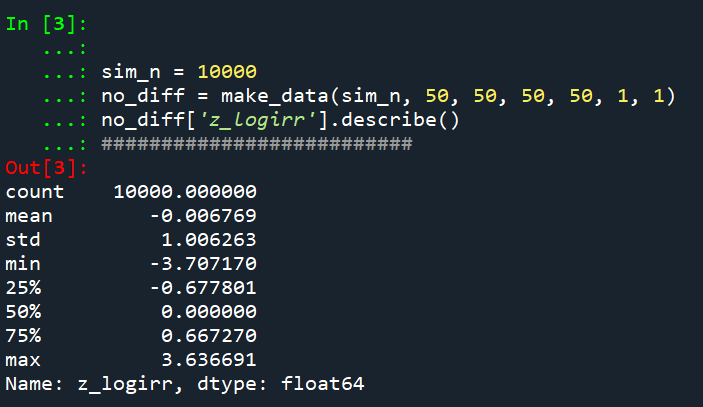So lets look at the scenario where the control area doesn’t change, but the treated area goes from 50 to 30. We can see again the point estimate in this scenario is spot on the money.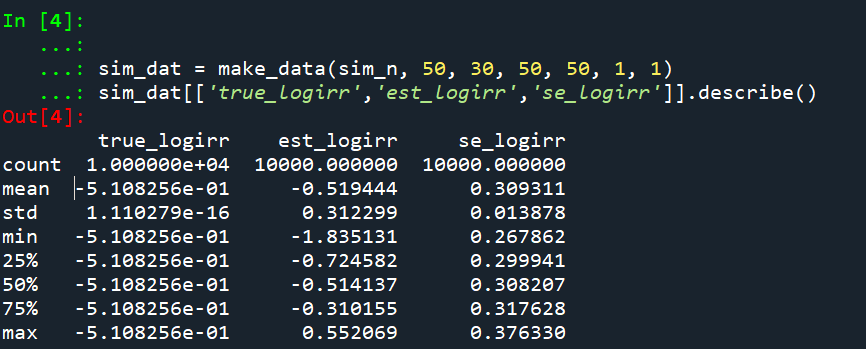And then we can see the coverage of the logged irr estimator is spot on as well: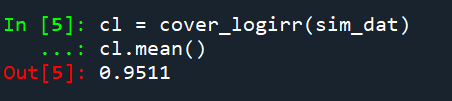So if you are interested in slightly different baseline scenarios, you can use that same simulation code to check out the behavior of David’s estimator and conduct simulated power analysis the same way I have shown for the WDD estimator in prior blog posts.

So if both are unbiased and have good coverage again, why would you prefer the WDD estimator over the IRR estimator (or vice-versa)? Well, lets take the 35% reduction I talked about at the beginning of the post, and the department needs to spend \$250k on extra officers to conduct whatever hot spot policing intervention. A 35% reduction may be worth it if we start with a baseline of 200 crimes (so would expect to go down to 130, for a reduction of 70 crimes). If the baseline is 20 crimes, it goes down to 13 crimes (so only a reduction of 7 crimes). The actual benefit of the IRR estimate is entirely dependent on the baseline count of crimes it is applied to.

Even if the IRR estimate is itself unbiased and has proper coverage, for even an individual study backing out the estimated reduction in total crimes from the IRR is biased. So here in this same simulated data (50 to 30 in treated, and 50 to 50 in control areas). The true count reduction is -20, and here is the point estimate on the X axis and the length of the confidence interval for each simulation on the Y axis for my WDD test. You can see they are nicely centered on -20, and the length of the confidence intervals has a very tiny variance – they are mostly just a smidge over 50 in total length. So that is probably tough to wrap your head around, but the variance of the variance estimates for the WDD are small.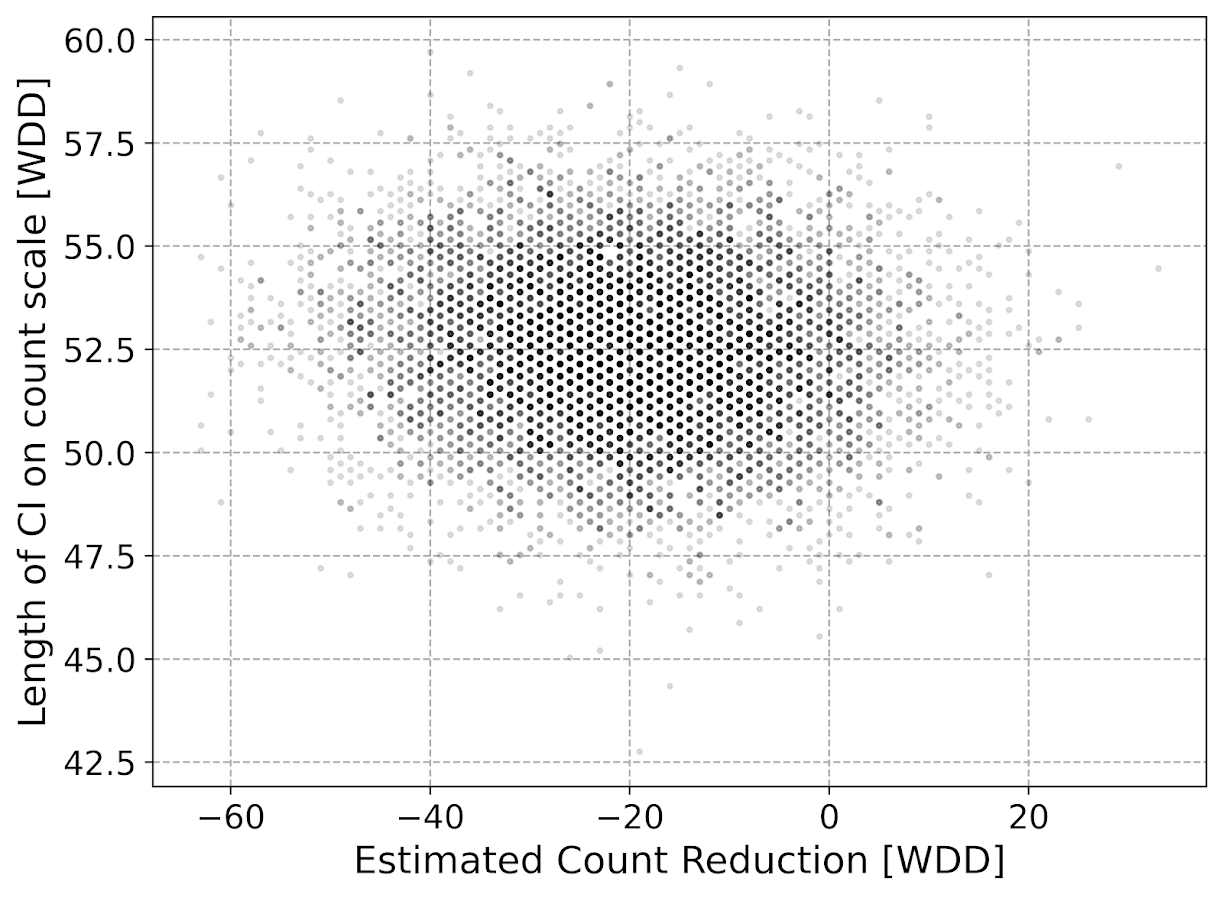Now lets do the same graph for the IRR estimate, but translated back out to a count crime reduction based on the simulated values: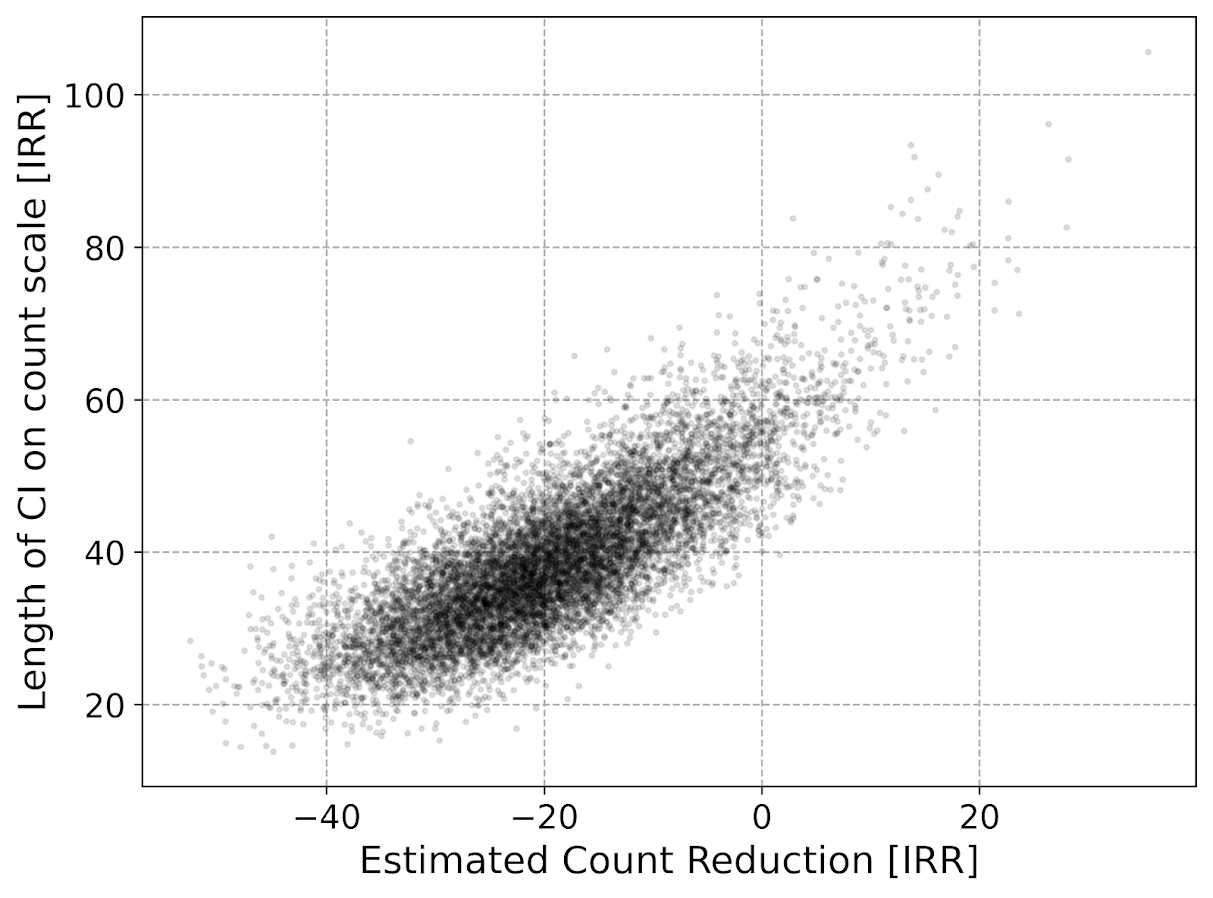We either have a ton of bias in this estimate (if the estimate of the count reduction is too large, the confidence interval is too small). Or the opposite, the estimate of the count reduction is too small, and the confidence interval is crazy wide. In Andrew Gelman’s terminology, it can result in pretty large type M (magnitude) errors in this simulated example (Gelman & Carlin, 2014). So the variance of the variance estimates in this scenario are quite large.

To be clear – if you are interested in estimating a percent reduction, by all means use David’s IRR estimator. If you however want to translate that percent reduction into an estimate of the total crimes reduced though you should use my WDD estimator in that case. You should not back out a total crimes reduced estimate from the IRR.

# Final Thoughts

So I have said a few times I think the IRR estimator makes more sense for meta-analyses. Why do I think that? Well, imagine we have an underlying causal process through which a hot spots policing experiment can randomly deter/prevent a particular proportion of crimes. That underlying causal process suggests an IRR effect. And also the problem I mention with translating back to crime counts I believe should get smaller with tighter estimates.

For a causal process that is more akin to my WDD estimator, imagine some crimes will always be deterred/prevented from a hot spots policing experiment, and some will never be. And we don’t know up-front which is which, so the observed reduction is based on whatever mixture of the two we have at that particular location.

The proportion reduction seems to make more sense to me for active patrol type interventions (which are ephemeral) vs permanent CPTED like interventions which should prevent certain criminal acts in perpetuity. But of course any situation in the real world could have both occurring at the same time.

When you go and look at the meta-analysis of hot spots policing, those interventions are all over the place (Hinkle et al., 2020). I think my WDD estimate would not make sense to mash up into a final meta-analytic estimate. The IRR may not make sense either in the end, but it is plausibly more relevant to compare the IRRs from a study with a baseline of 200 crimes vs one with 40 crimes at baseline. I am not sure it makes sense to compare WDDs in that scenario. But that being said, a few of my blog posts have discussed the WDD normalized per unit area or per unit time. Those normalized estimates are probably more apples to apples in the 200 vs 40 scenario.

A final note I have not discussed here is that David discusses a correction for overdispersion, so that is a potential feather in the cap for his estimator vs the WDD. I’d be a bit hesitant though with that – only four observations to estimate the dispersion term is slicing it a bit thin IMO. But I was wrong about the original estimator, so I may be wrong about that as well. It will take simulation evidence to determine that though – David’s paper just provides the correction term, he doesn’t provide evidence for its utility with small sample data.

And to be fair I have not done simulations to see how my estimator behaves in the presence of overdispersion either. I believe it will simply just cause the standard errors to be too small, so like in Wheeler (2016), I imagine it will just require upping the interval (e.g. use a z-score of 3 instead of 2) to get proper coverage for real crime data.

# References

Other Posts of Interest

# Python simulation code

Here is a copy-pasted chunk of the entire python simulation code.

``````'''
Comparing WDD to log(IRR) from Wilson's

Andy Wheeler
'''

import pandas as pd
import numpy as np
from scipy.stats import norm
from scipy.stats import poisson
from scipy.stats import uniform
import matplotlib
import matplotlib.pyplot as plt
import os
my_dir = r'D:\Dropbox\Dropbox\Documents\BLOG\wdd_vs_irr'
os.chdir(my_dir)

#########################################################
#Settings for matplotlib

andy_theme = {'axes.grid': True,
'grid.linestyle': '--',
'legend.framealpha': 1,
'legend.facecolor': 'white',
'legend.fontsize': 14,
'legend.title_fontsize': 16,
'xtick.labelsize': 14,
'ytick.labelsize': 14,
'axes.labelsize': 16,
'axes.titlesize': 20,
'figure.dpi': 100}

matplotlib.rcParams.update(andy_theme)
#########################################################

#This works for the scipy functions as well
np.random.seed(seed=10)

# A function to generate the WDD estimate for simulated data
def wdd_sim(treat0,treat1,cont0,cont1,pre,post):
tr_cr_0 = poisson.rvs(mu = treat0, size=int(pre)).sum()
co_cr_0 = poisson.rvs(mu = cont0, size=int(pre)).sum()
tr_cr_1 = poisson.rvs(mu = treat1, size=int(post)).sum()
co_cr_1 = poisson.rvs(mu = cont1, size=int(post)).sum()
# WDD estimates
est = ( tr_cr_1/post - tr_cr_0/pre ) - ( co_cr_1/post - co_cr_0/pre )
post2 = (1/post)**2
pre2 = (1/pre)**2
var_est = tr_cr_0*pre2 + tr_cr_1*post2 + co_cr_0*pre2 + co_cr_1*post2
true_val = ( treat1 - treat0 ) - ( cont1 - cont0 )
z_score = est / np.sqrt(var_est)
# Wilson log IRR estimates
true_logirr = np.log( (treat1*cont0) / (cont1*treat0) )
est_logirr = np.log( ((tr_cr_1/post)*(co_cr_0/pre)) / ( (co_cr_1/post)*(tr_cr_0/pre) ) )
se_logirr = np.sqrt( 1/tr_cr_1 + 1/co_cr_0 + 1/co_cr_1 + 1/tr_cr_0 )
z_logirr = est_logirr / se_logirr
return (tr_cr_0, co_cr_0, tr_cr_1, co_cr_0, est, var_est, true_val, z_score, true_logirr, est_logirr, se_logirr, z_logirr)

def make_data(n, treat0, treat1, cont0, cont1, pre, post):
base = pd.DataFrame( range(n), columns=['index'])
base['treat0'] = treat0
if treat1 is not None:
base['treat1'] = treat1
else:
base['treat1'] = base['treat0']
if cont0 is not None:
base['cont0'] = cont0
else:
base['cont0'] = base['treat0']
if cont1 is not None:
base['cont1'] = cont1
else:
base['cont1'] = base['cont0']
base.drop(columns='index',inplace=True)
base['pre'] = pre
base['post'] = post
sim_vals = base.apply(lambda x: wdd_sim(**x), axis=1, result_type='expand')
sim_vals.columns = ['sim_t0','sim_c0','sim_t1','sim_c1','est','var_est','true_val','z_score',
'true_logirr','est_logirr','se_logirr','z_logirr']
return pd.concat([base,sim_vals], axis=1)

# Coverage of the log irr estimate
# Lets look at the coverage rate for a decline from 40 to 20
def cover_logirr(data, ci=0.95):
mult = (1 - ci)/2
nv = norm.ppf(1 - mult)
dif = nv*data['se_logirr']
low = data['est_logirr'] - dif
high = data['est_logirr'] + dif
cover = ( data['true_logirr'] > low) & ( data['true_logirr'] < high )
return cover

# Length of ci for WDD
def len_ci(data, ci=0.95):
mult = (1 - ci)/2
nv = norm.ppf(1 - mult)
dif = nv*np.sqrt( data['var_est'] )
low = data['est'] - dif
high = data['est'] + dif
return low, high, high - low

# Length of ci for IRR estimate on count scale
# This depends on the baseline estimate to multiply
# The IRR by, using the baseline average of the
# Treatment area

def len_irr(data, ci=0.95):
mult = (1 - ci)/2
nv = norm.ppf(1 - mult)
dif = nv*data['se_logirr']
low = data['est_logirr'] - dif
high = data['est_logirr'] + dif
baseline = data['sim_t0']/data['pre']
# Even if you use hypothetical, the variance is quite high
#baseline = data['treat0']
est_count = baseline*np.exp(data['est_logirr']) - baseline
c1 = baseline*np.exp(low) - baseline
c2 = baseline*np.exp(high) - baseline
return est_count, c1, c2, np.abs(c2 - c1)

##########################
# Example with no change, lets look at the null distribution
sim_n = 10000
no_diff = make_data(sim_n, 50, 50, 50, 50, 1, 1)
no_diff['z_logirr'].describe()
##########################

##########################
# Example with equal time periods, a reduction from 50 to 30 and 50 to 50 in control area
sim_dat = make_data(sim_n, 50, 30, 50, 50, 1, 1)
sim_dat[['true_logirr','est_logirr','se_logirr']].describe()

cl = cover_logirr(sim_dat)
cl.mean()

# Compare length of CI for IRR vs WDD

# WDD length
lowdd, highwdd, lwdd = len_ci(sim_dat)
lwdd.describe()

# IRR length on the count scale
est_cnt_irr, lo_irr, hi_irr, ln_irr = len_irr(sim_dat)
ln_irr.describe()

# Scatterplot of estimated count reduction vs
# Length of CI
fig, ax = plt.subplots(figsize=(8,6))
ax.scatter(est_cnt_irr, ln_irr, c='k',
alpha=0.1, s=4)
ax.set_axisbelow(True)
ax.set_xlabel('Estimated Count Reduction [IRR]')
ax.set_ylabel('Length of CI on count scale [IRR]')
plt.savefig('IRR_Len_Est.png', dpi=500, bbox_inches='tight')
plt.show()

# Lets compare to the WDD estimate
fig, ax = plt.subplots(figsize=(8,6))
ax.scatter(sim_dat['est'], lwdd, c='k',
alpha=0.1, s=4)
ax.set_axisbelow(True)
ax.set_xlabel('Estimated Count Reduction [WDD]')
ax.set_ylabel('Length of CI on count scale [WDD]')
plt.savefig('WDD_Len_Est.png', dpi=500, bbox_inches='tight')
plt.show()
##########################``````

# Simulating Group Based Trajectories (in R)

The other day I pointed out on Erwin Kalvelagen’s blog how mixture models are a solution to fit regression models with multiple lines (where identification of which particular function/line is not known in advance).

I am a big fan of simulating data when testing out different algorithms for simply the reason it is often difficult to know how an estimator will behave with your particular data. So we have a bunch of circumstances with mixture models (in particular here I am focusing on repeated measures group based traj type mixture models) that it is hard to know upfront how they will do. Do you want to estimate group based trajectories, but have big N and small T? Or the other way, small N and big T? (Larger sample sizes tend to result in identifying more mixtures as you might imagine (Erosheva et al., 2014).) Do you have sparse Poisson data? Or high count Poisson data? Do you have 100,000 data points, and want to know how big of data and how long it may take? These are all good things to do a simulation to see how it behaves when you know the correct answer.

These are relevant no matter what the particular algorithm – so the points are all the same for k-medoids for example (Adepeju et al., 2021; Curman et al., 2015). Or whatever clustering algorithm you want to use in this circumstance. So here I show a few different simulations showing:

• GBTM can recover the correct underlying equations
• AIC/BIC fit stats have a difficult time distinguishing the correct number of groups
• If the underlying model is a random effects instead of latent clusters, AIC/BIC performs quite well

The last part is because GBTM models have a habit of spitting out solutions, even if the true underlying data process has no discrete groups. This is what Skardhamar (2010) did in his article. It was focused on life course, but it applies equally to the spatial analysis GBTM myself and others have done as well (Curman et al., 2015; Weisburd et al., 2004; Wheeler et al., 2016). I’ve pointed out in the past that even if the fit for GBTM looks good, the underlying data can suggest a random effects model will work quite well, and Greenberg (2016) makes pretty much the same point as well.

# Example in R

In the past I have shown how to use the crimCV package to fit these group based traj models, specifically zero-inflated Poisson models (Nielsen et al., 2014). Here I will show a different package, the R `flexmix` package (Grün & Leisch, 2007). This will be Poisson mixtures, but they have an example of doing zip models in there docs if you want.

So first, I load in the `flexmix` library, set the seed, and generate longitudinal data for three different Poisson models. One thing to note here, mixture models don’t assign an observation 100% to an underlying mixture, but the data I simulate here is 100% in a particular group.

``````################################################
library("flexmix")
set.seed(10)

# Generate simulated data
n <- 200 #number of individuals
t <- 10   #number of time periods
dat <- expand.grid(t=1:t,id=1:n)

# Setting up underlying 3 models
time <- dat\$t
p1 <- 3.5 - time
p2 <- 1.3 + -1*time + 0.1*time^2
p3 <- 0.15*time
p_mods <- data.frame(p1,p2,p3)

# Selecting one of these by random
# But have different underlying probs
latent <- sample(1:3, n, replace=TRUE, prob=c(0.35,0.5,0.15))
dat\$lat <- expand.grid(t=1:t,lat=latent)\$lat
dat\$sel_mu <- p_mods[cbind(1:(n*t), dat\$lat)]
dat\$obs_pois <- rpois(n=n*t,lambda=exp(dat\$sel_mu))
################################################``````

Now that is the hard part really – figuring out exactly how you want to simulate your data. Here it would be relatively simple to increase the number of people/areas or time period. It would be more difficult to figure out underlying polynomial functions of time.

Next part we fit a 3 mixture model, then assign the highest posterior probabilities back into the original dataset, and then see how we do.

``````################################################
# Now fitting flexmix model
mod3 <- flexmix(obs_pois ~ time + I(time^2) | id,
model = FLXMRglm(family = "poisson"),
data = dat, k = 3)
dat\$mix3 <- clusters(mod3)

# Seeing if they overlap with true labels
table(dat\$lat, dat\$mix3)/t
################################################``````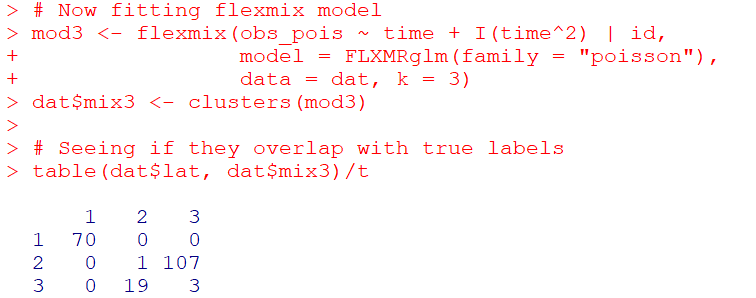So you can see that the identified groupings are quite good. Only 4 groups out of 200 are mis-placed in this example.

Next we can see if the underlying equations were properly recovered (you can have good separation between groups, but the polynomial fit may be garbage).

``````# Seeing if the estimated functions are close
rm3 <- refit(mod3)
summary(rm3)``````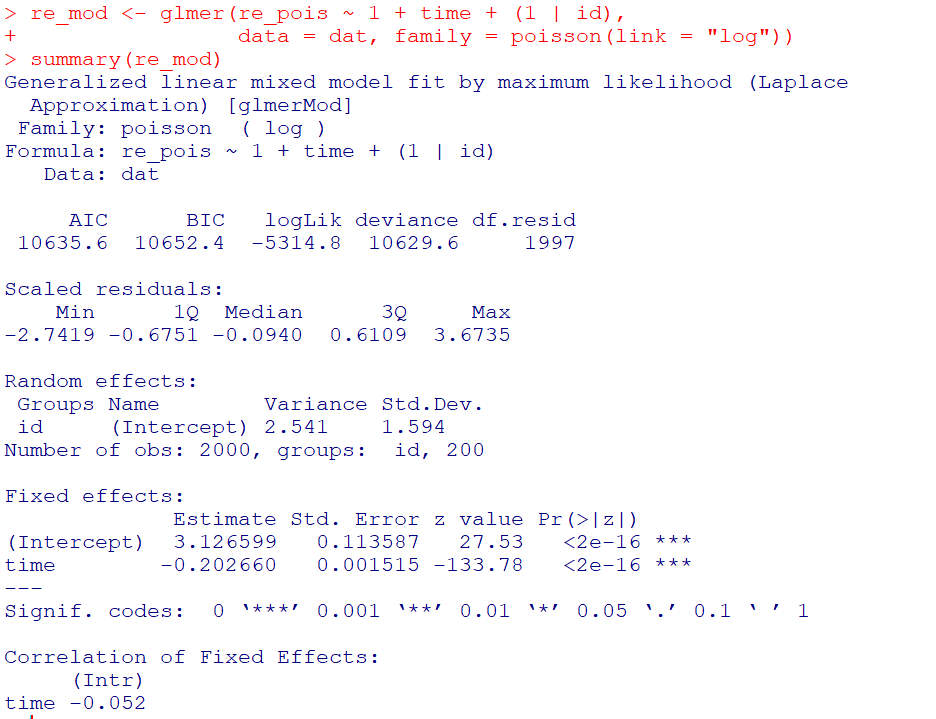This shows the equations are really as good as you could expect. The standard errors are as wide as they are because this isn’t really all that large a data sample for generalized linear models.

So this shows that if I feed in the correct underlying equation (almost, I could technically submit different equations with/without quadratic terms for example). But what about the real world situation in which you do not know the correct number of groups? Here I fit models for 1 to 8 groups, and then use the typical AIC/BIC to see which group it selects:

``````################################################
# If I look at different groups will AIC/BIC
# pick the right one?

group <- 1:8
left_over <- group[!(group %in% 3)]
aic <- rep(-1, 8)
bic <- rep(-1, 8)
aic <- AIC(mod3)
bic <- BIC(mod3)

for (i in left_over){
mod <- flexmix(obs_pois ~ time + I(time^2) | id,
model = FLXMRglm(family = "poisson"),
data = dat, k = i)
aic[i] <- AIC(mod)
bic[i] <- BIC(mod)
}

fit_stats <- data.frame(group,aic,bic)
fit_stats
################################################``````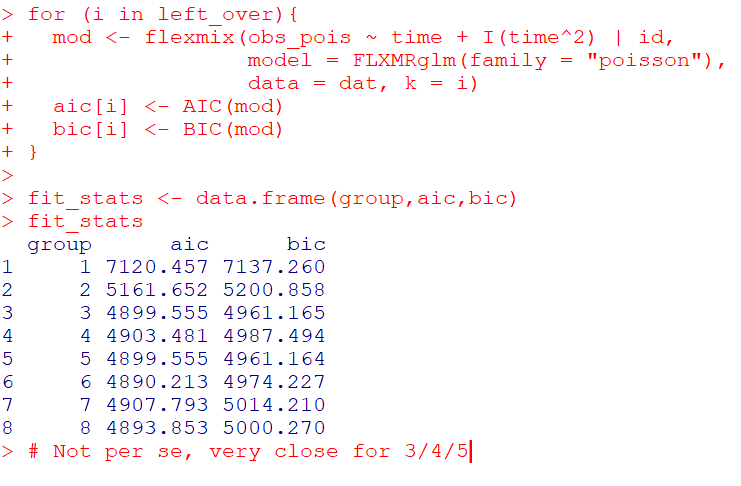Here it actually fit the same model for 3/5 groups (sometimes even if you tell flexmix to fit 5 groups, it will only return a smaller number). You can see that the fit stats for group 4 through are almost the same. So while AIC/BIC did technically pick the right number in this simulated example, it is cutting the margin pretty close to picking 4 groups in this data instead of 3.

So the simulation Skardhamar (2010) did was slightly different than this so far. What he did was simulate data with no underlying trajectory groups, and then showed GBTM tended to spit out solutions. Here I will show that is the case as well. I simulate random intercepts and a simple linear trend over time.

``````################################################
# Simulate random effects model
library(lme4)
rand_eff <- rnorm(n=n,0,1.5)
dat\$re <- expand.grid(t=1:t,re=rand_eff)\$re
dat\$re_pois <- rpois(n=n*t,lambda=exp(dat\$sel_mu))
dat\$mu_re <- 3 + -0.2*time + dat\$re
dat\$re_pois <- rpois(n=n*t,lambda=exp(dat\$mu_re))

re_mod <- glmer(re_pois ~ 1 + time + (1 | id),
data = dat, family = poisson(link = "log"))
summary(re_mod)
################################################``````So you can see that the random effects model is all fine and dandy – recovers both the fixed coefficients, as well as estimates the correct variance for the random intercepts.

So here I go and see how the AIC/BIC compares for the random effects models vs GBTM models for 1 to 8 groups (I stuff the random effects model in the first row for group 0):

``````################################################
# Test AIC/BIC for random effects vs GBTM
group <- 0:8
left_over <- 1:8
aic <- rep(-1, 9)
bic <- rep(-1, 9)
aic <- AIC(re_mod)
bic <- BIC(re_mod)

for (i in left_over){
mod <- flexmix(re_pois ~ time + I(time^2) | id,
model = FLXMRglm(family = "poisson"),
data = dat, k = i)
aic[i+1] <- AIC(mod)
bic[i+1] <- BIC(mod)
}

fit_stats <- data.frame(group,aic,bic)
fit_stats
################################################``````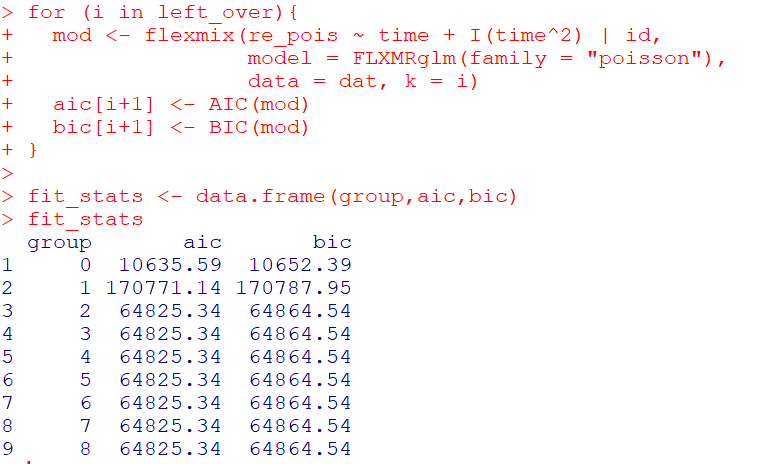So it ends up flexmix will not give us any more solutions than 2 groups. But that the random effect fit is so much smaller (either by AIC/BIC) than the GBTM you wouldn’t likely make that mistake here.

I am not 100% sure how well we can rely on AIC/BIC for these different models (R does not count the individual intercepts as a degree of freedom here, so k=3 instead of k=203). But no reasonable accounting of k would flip the AIC/BIC results for these particular simulations.

One of the things I will need to experiment with more, I really like the idea of using out of sample data to validate these models instead of AIC/BIC – no different than how Nielsen et al. (2014) use leave one out CV. I am not 100% sure if that is possible in this set up with `flexmix`, will need to investigate more. (You can have different types of cross validation in that context, leave entire groups out, or forecast missing data within an observed group.)

# References

Adepeju, M., Langton, S., & Bannister, J. (2021). Anchored k-medoids: a novel adaptation of k-medoids further refined to measure long-term instability in the exposure to crime. Journal of Computational Social Science, 1-26.

Grün, B., & Leisch, F. (2007). Fitting finite mixtures of generalized linear regressions in R. Computational Statistics & Data Analysis, 51(11), 5247-5252.

Curman, A. S., Andresen, M. A., & Brantingham, P. J. (2015). Crime and place: A longitudinal examination of street segment patterns in Vancouver, BC. Journal of Quantitative Criminology, 31(1), 127-147.

Erosheva, E. A., Matsueda, R. L., & Telesca, D. (2014). Breaking bad: Two decades of life-course data analysis in criminology, developmental psychology, and beyond. Annual Review of Statistics and Its Application, 1, 301-332.

Greenberg, D. F. (2016). Criminal careers: Discrete or continuous?. Journal of Developmental and Life-Course Criminology, 2(1), 5-44.

Nielsen, J. D., Rosenthal, J. S., Sun, Y., Day, D. M., Bevc, I., & Duchesne, T. (2014). Group-based criminal trajectory analysis using cross-validation criteria. Communications in Statistics-Theory and Methods, 43(20), 4337-4356.

Skardhamar, T. (2010). Distinguishing facts and artifacts in group-based modeling. Criminology, 48(1), 295-320.

Weisburd, D., Bushway, S., Lum, C., & Yang, S. M. (2004). Trajectories of crime at places: A longitudinal study of street segments in the city of Seattle. Criminology, 42(2), 283-322.

Wheeler, A. P., Worden, R. E., & McLean, S. J. (2016). Replicating group-based trajectory models of crime at micro-places in Albany, NY. Journal of Quantitative Criminology, 32(4), 589-612.

# Weekly Error Bar Chart in Tableau

I have posted my Tableau tutorial #2 for making a weekly error bar chart in Tableau. (Tutorial #1 was for a seasonal chart.) This is replicating prior examples I provided in Excel for IACA workshops and my undergrad crime analysis course.

WEEKLY ERROR BAR CHART TUTORIAL

For a sneak peak of the end result, see here:Making error bars in Tableau is quite a chore. One approach that people use for Excel, making a cumulative area chart, and then make the under area invisible, does not work in Tableau. Since you can interact with everything, making something that is there but invisible is not an option. You could do that approach and turn the area white, but then the gridlines or anything below that object are not visible.

So the best workaround I found here was to do discrete time, and use the reference band option in the background. This is a good example for non-normal error bars, here this is for low count Poisson data, but another use case I will have to show sometime are for proportion confidence intervals in Tableau. (This is one reason I am doing this, I need to do something similar for my work for proportions to monitor my machine learning models. No better way to teach myself than to do it myself.)

Next up I will have to show an example that illustrates the unique ability of Tableau (at least relative to Excel) – making a dashboard that has brushing/linking. Tinkering with showing that off using this same example data with a geographic map as well. My dashboards I have tried so far all tend to look not very nice though, so I will need to practice some more before I can show those off.

# The WDD test with different area sizes

So I have two prior examples of weighting the WDD test (a simple test for pre-post crime counts in an experimental setting):

And a friend recently asked about weighting for different areas, so the test is crime reduction per area density instead of overall counts. First before I get into the example, this isn’t per se necessary. All that matters in the end for this test to be valid is 1) the crime data are Poisson distributed, 2) the control areas follow parallel trends to the treated area. So based on this I’ve advocated that it is ok to have a control area be ‘the rest of the city’ for example.

Some of my work on long term crime trends at micro places, shows low-crime and high-crime areas all tend to follow the same overall temporal trends (and Martin Andresen’s related work one would come to the same conclusion). So that would suggest you can aggregate up many low crimes to make a reasonable control comparison to a hot spot treated area.

So as I will show weighting by area is possible, but it actually changes the identification strategy slightly (whereas the prior two weighting examples do not) – the parallel trends assumption needs to be on the crime per area estimate, as opposed to the original count scale. Since the friend who asked about this is an Excel GURU (check out Grant’s very nice YouTube videos for crime analysis) I will show how to do the calculations in Excel, as well as how to do a simulation to show my estimator behaves as it should. (And the benefit of that is you can do power analysis based on the simulations.)

# Example Calculations in Excel

I have posted an Excel spreadsheet to show the calculations here. But for a quick overview, I made the spreadsheet very similar to the original WDD calculation, you just need to insert your areas for the different treated/control/displacement areas.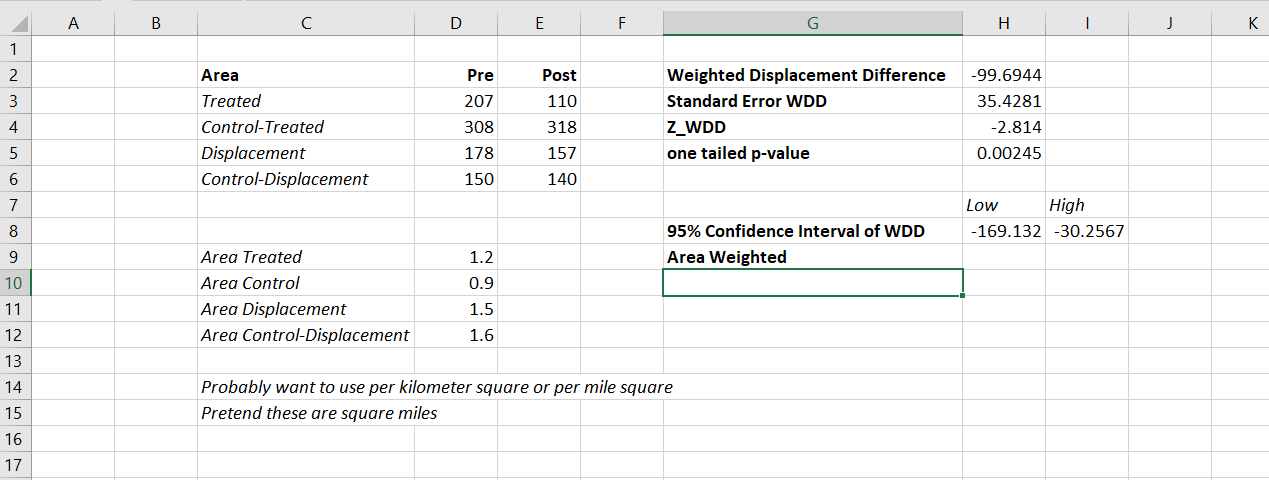And you can check out the formulas, again it is just weighting the estimator by the areas, and then making the appropriate transformations to the variance estimates.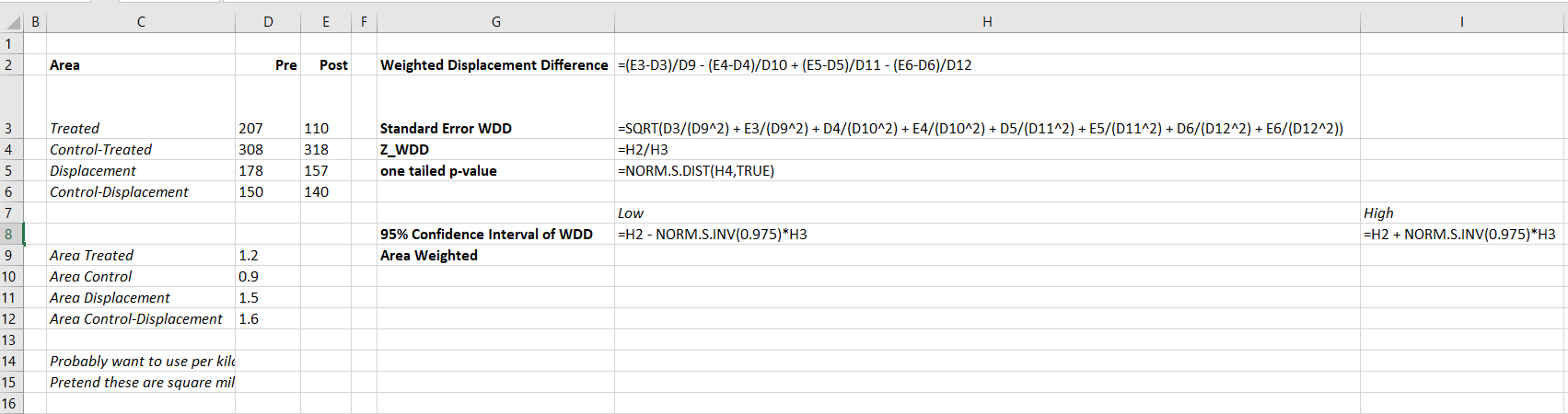I have an added extra portion of this though – a simulation tab to show the estimator works.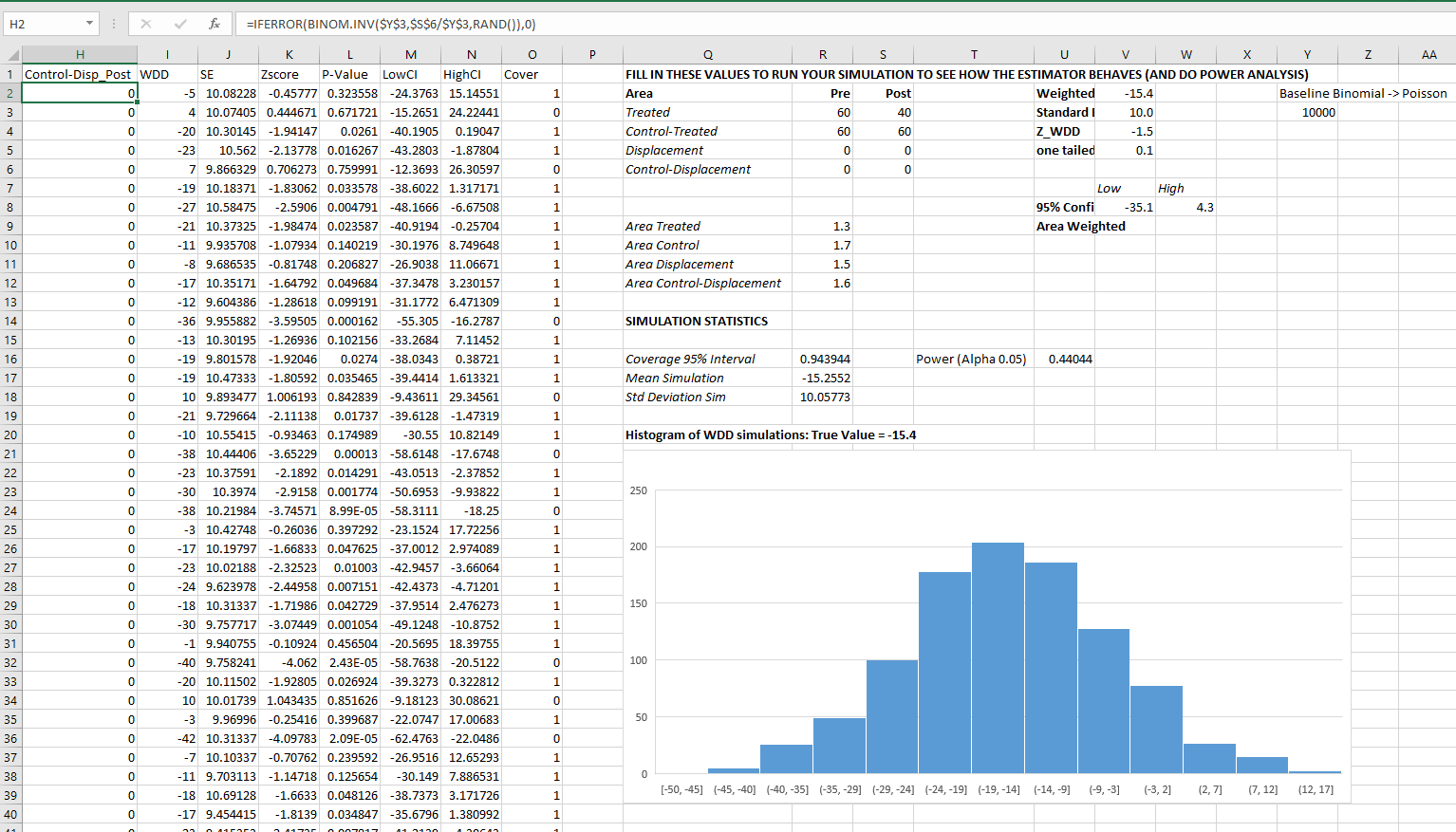Only thing to note, a way to simulate to Poisson data in Excel is to generate a random number on the unit interval `(0,1)`, and then for the distribution of interest use the inverse CDF function. There is no inverse Poisson function in Excel, but you can reasonably approximate it via the inverse binomial with a very large number of trials. I’ve tested and it is good enough for my purposes to use a base of 10k for the binomial trials.

The simulation tab on this spreadsheet you can input your own numbers for planning purposes as well. So the idea is if you think you can only reasonably reduce crimes by X amount in your targeted areas, this lets you do power analysis. So in this example, going from 60 to 40 crimes results in a power estimate of only 0.44 (so you will fail to reject the null over 5 out of 10 times, even if your intervention actually works as well as you think). But if you think you can reduce crimes from 60 to 30, the power in this example gets close to 0.8 (what you typically shoot for in up-front experiments, although there is no harm for going for higher power!). So if you have low power you may want to expand the time periods under study or expand the number of treated areas.

# Wrap Up

Between this and the prior WDD examples, I have about wrapped up all the potential permutations of weighting I can think of offhand. So you can mix/match all of these different weighting strategies together (e.g. you could do multiple time periods and area weighting). It is just algebra and carrying through the correct changes to the variance estimates.

I do have one additional blog post slated in the future. David Wilson has a recent JQC article using a different estimate, but essentially the same pre/post data I am using here. The identifying assumptions are different again for this (parallel trends on the ratio scale, not the linear scale), and I will have more to say when I think you would prefer the WDD to David’s estimator. (In short I think David’s is good for meta-analysis, but I prefer my WDD for individual evaluations.)